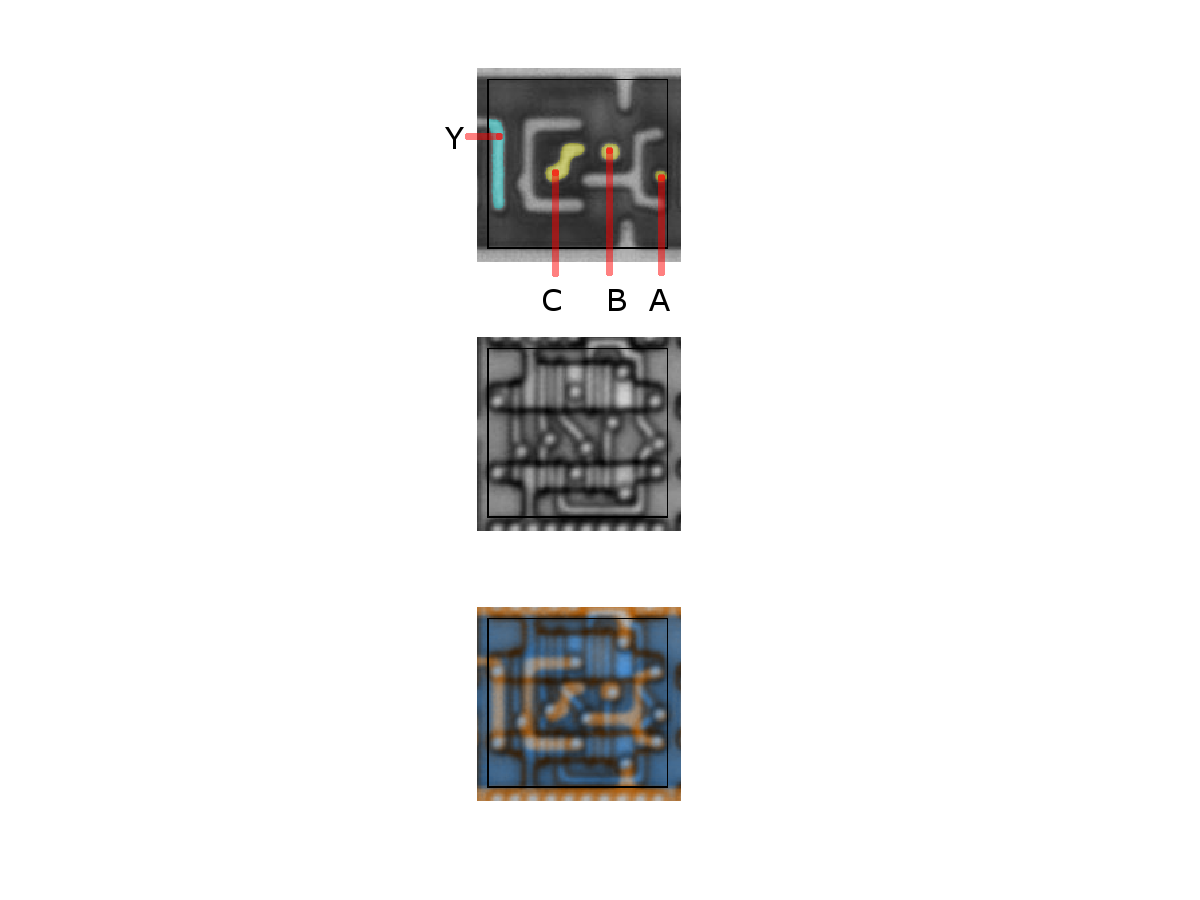# Inverter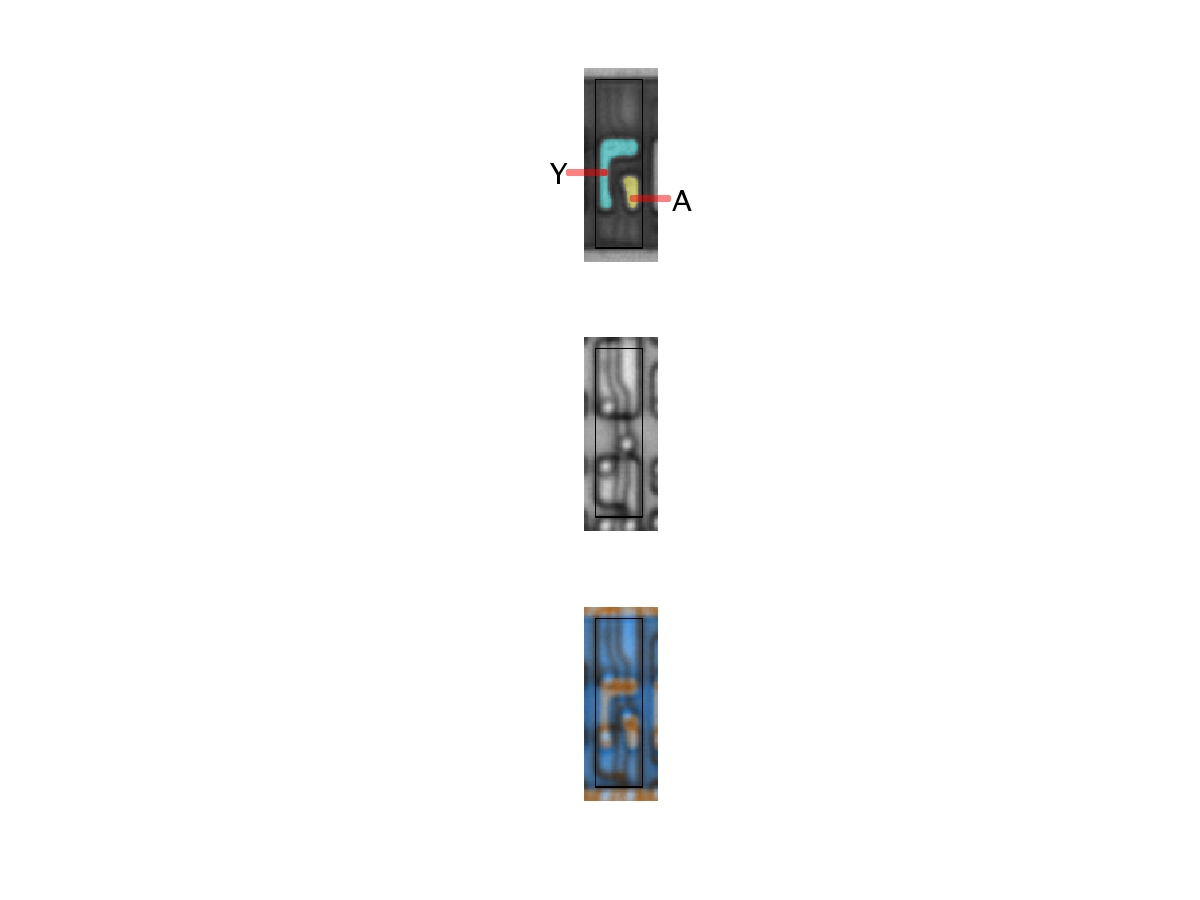inverter
Binary function: Y = ~Ainv
Binary function: Y = ~A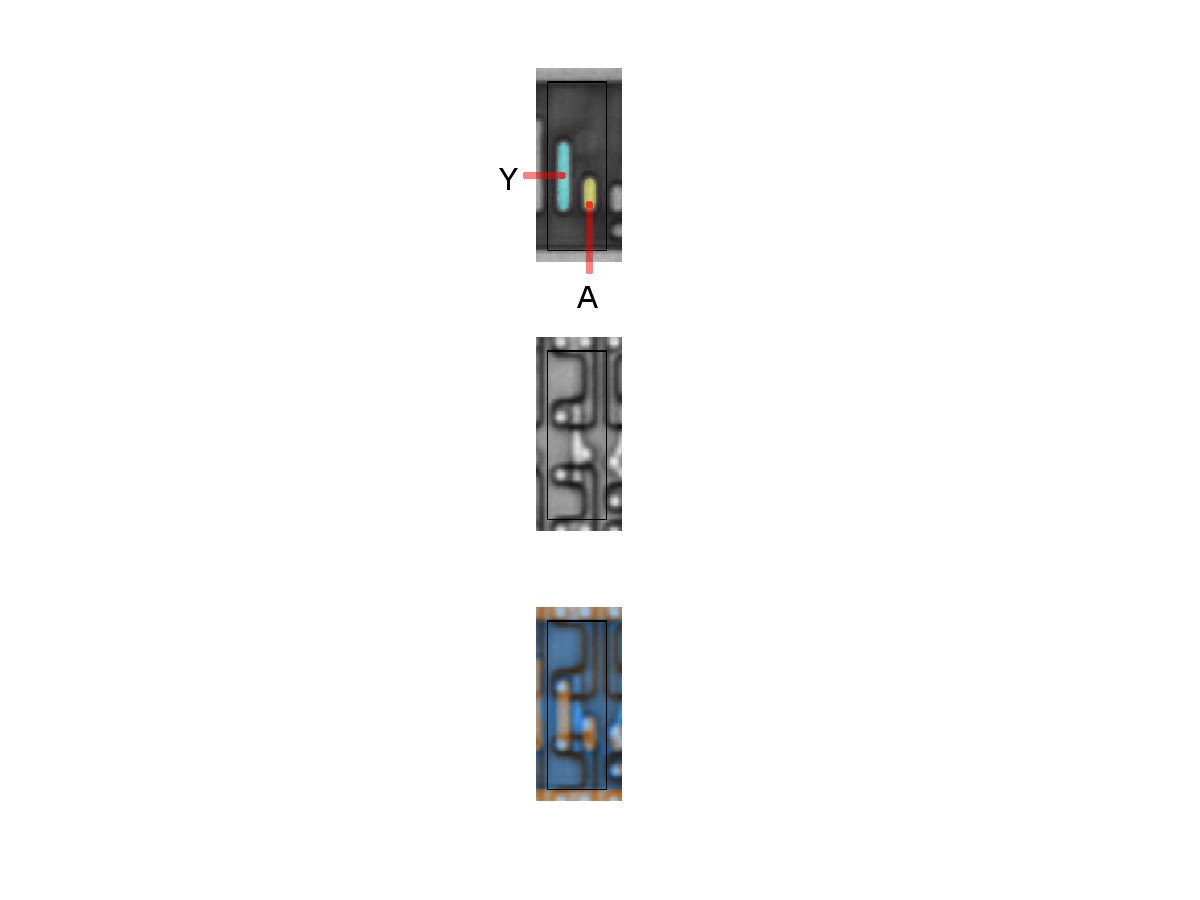inv
Binary function: Y = ~Ainv
Binary function: Y = ~Ainv
Binary function: Y = ~A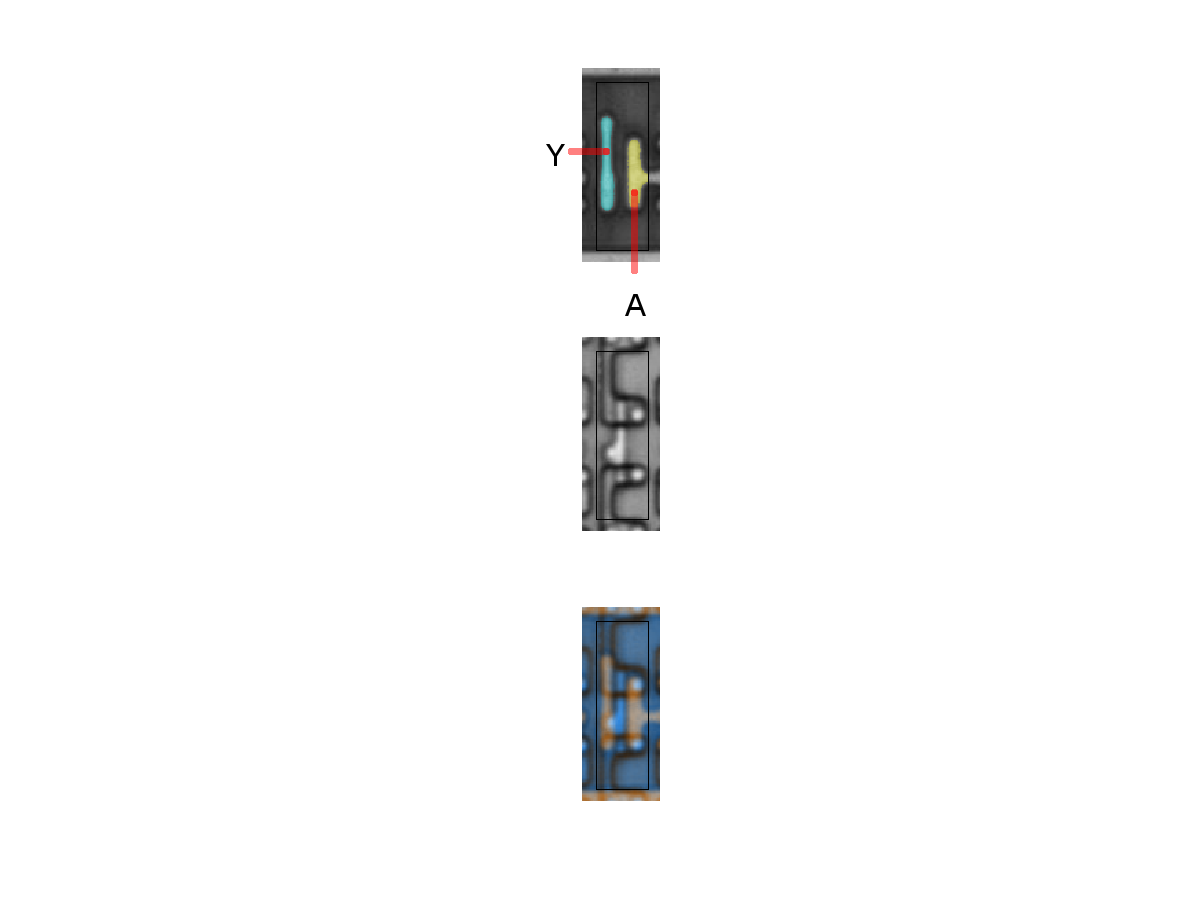inv
Binary function: Y = ~A

# Buffersbuffer
Binary function: Y = A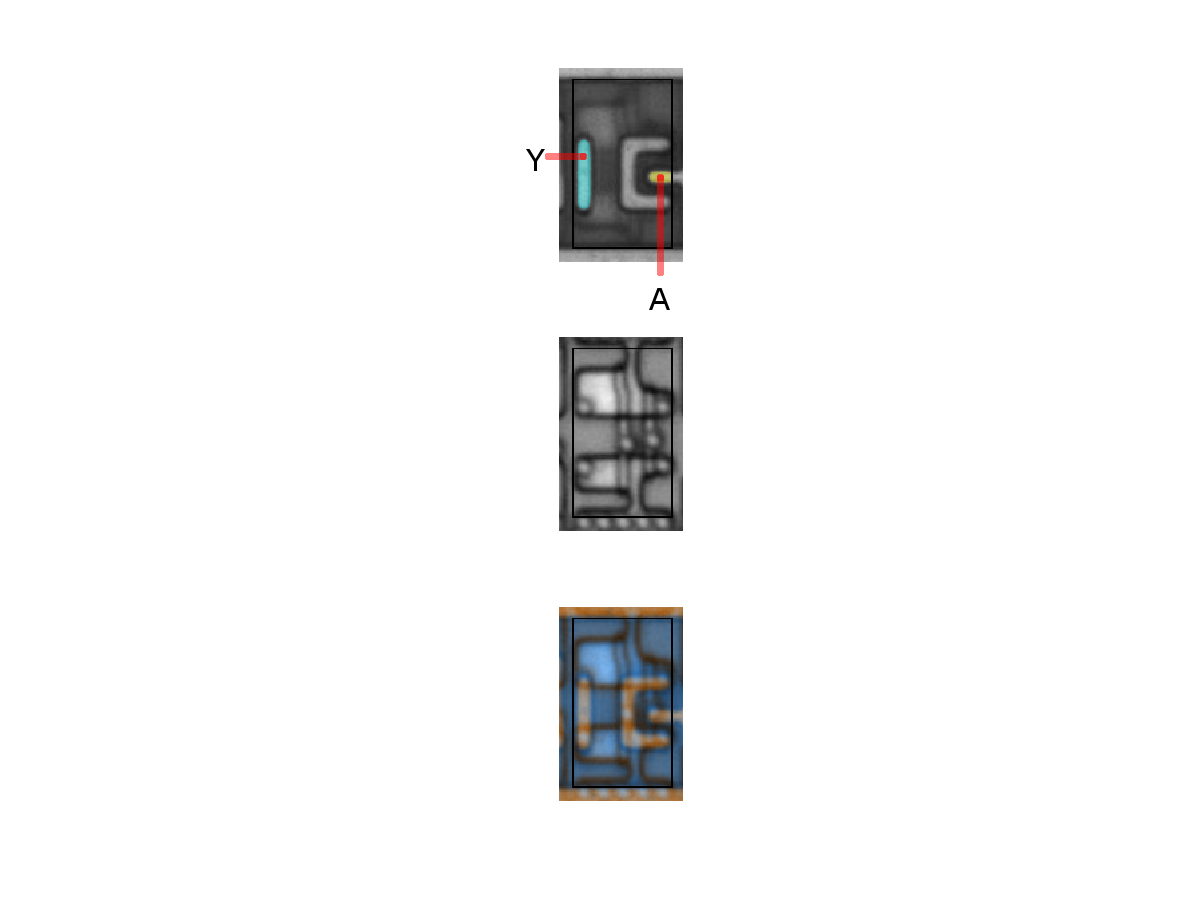buffer
Binary function: Y = ABuffer
Binary function: Y = A

# NANDs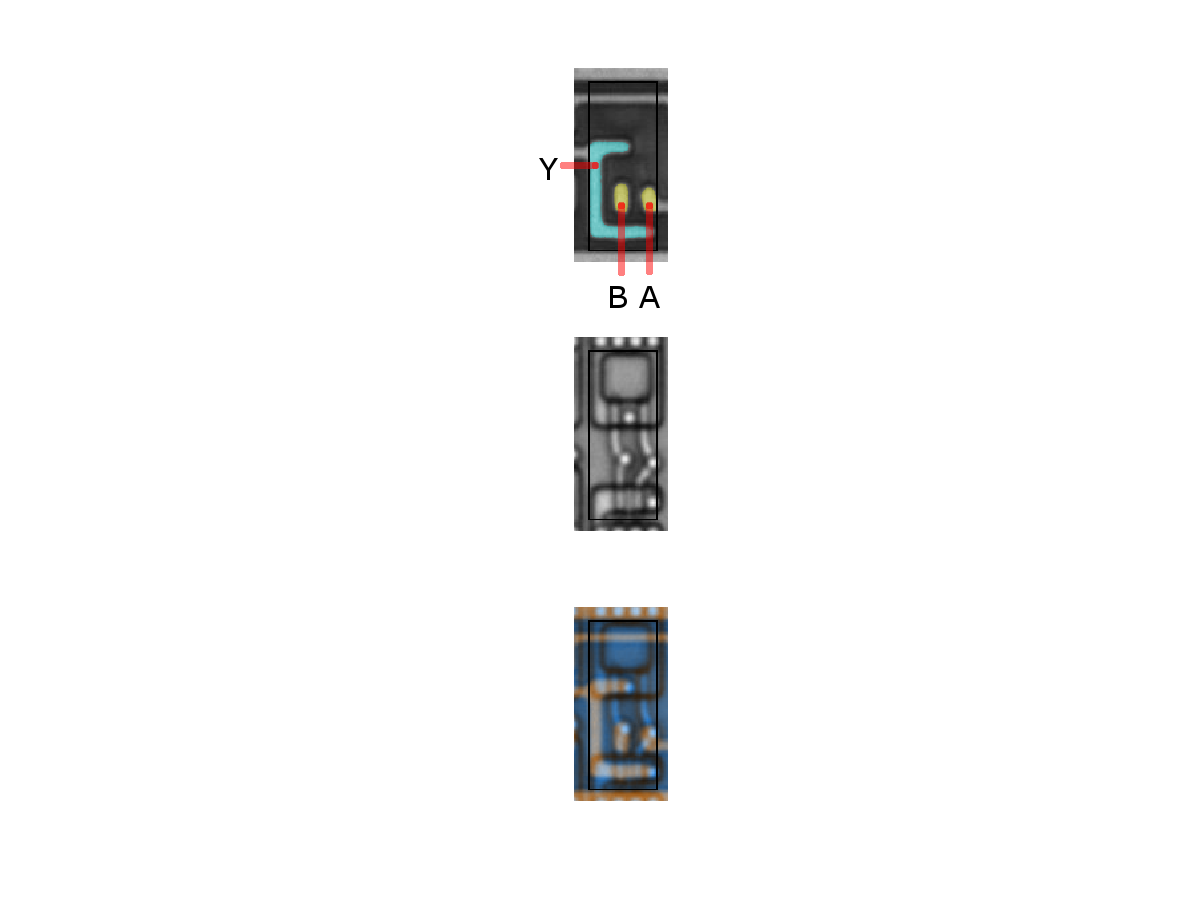2-NAND
Binary function: Y = ~(AB)2-NAND
Binary function: Y = ~( AB )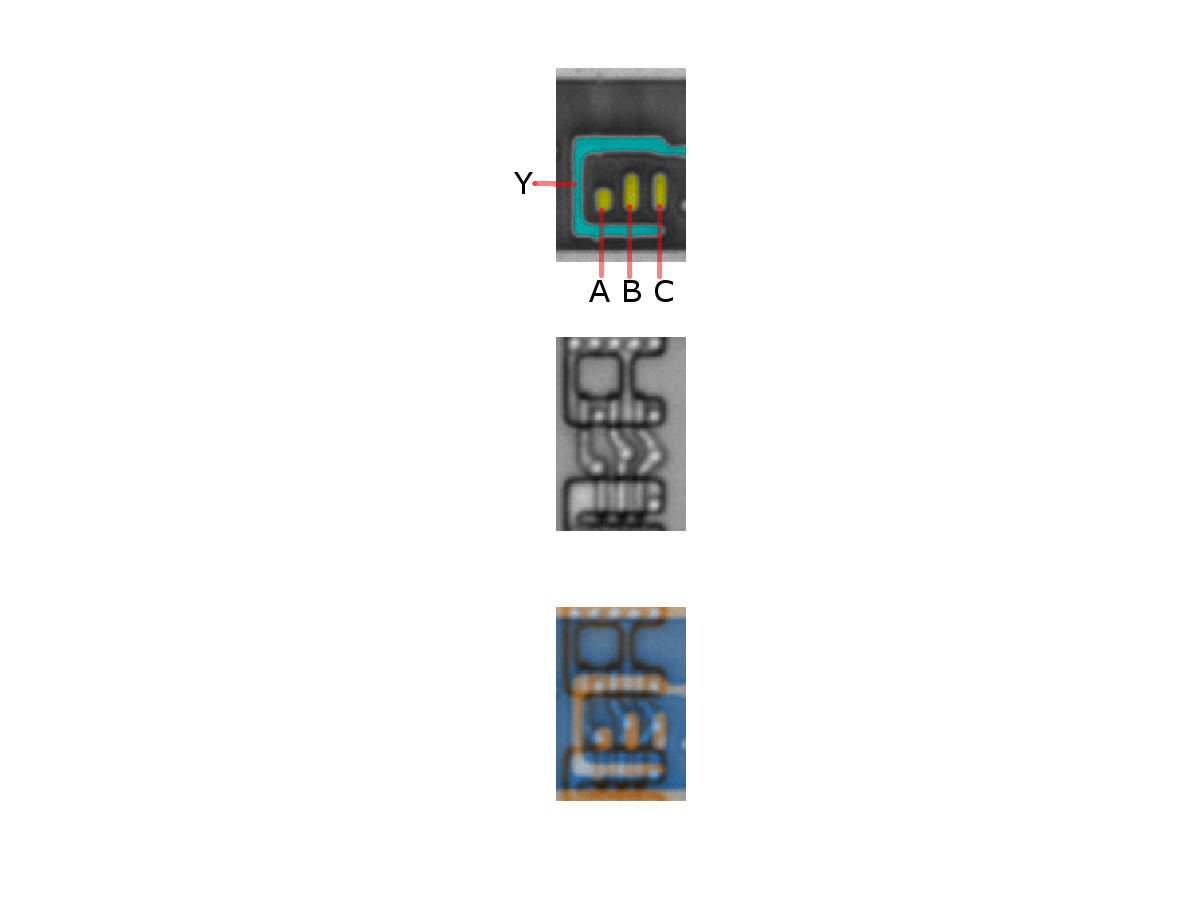3-NAND
Binary function: Y = ~( ABC )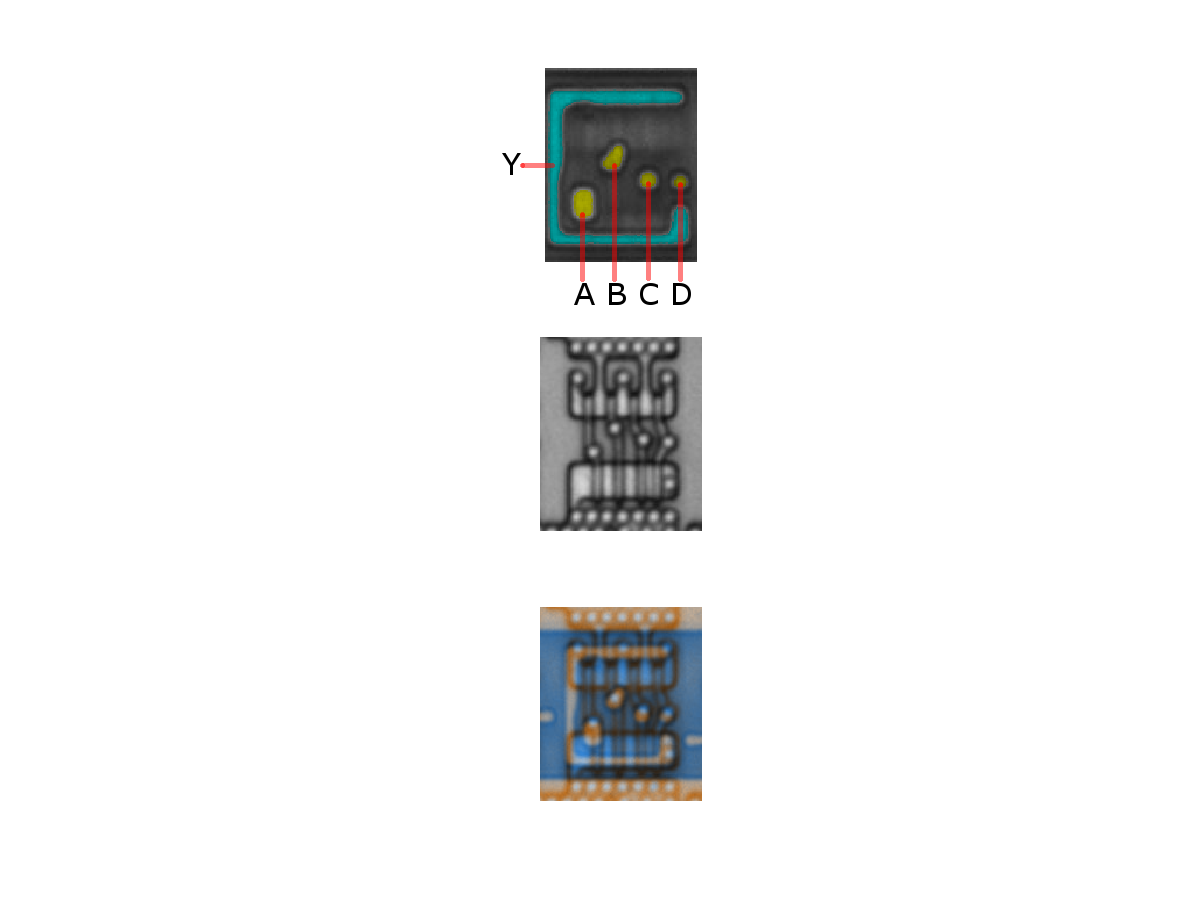4-NAND
Binary function: Y = ~( ABCD )

# (N)OR/X(N)OR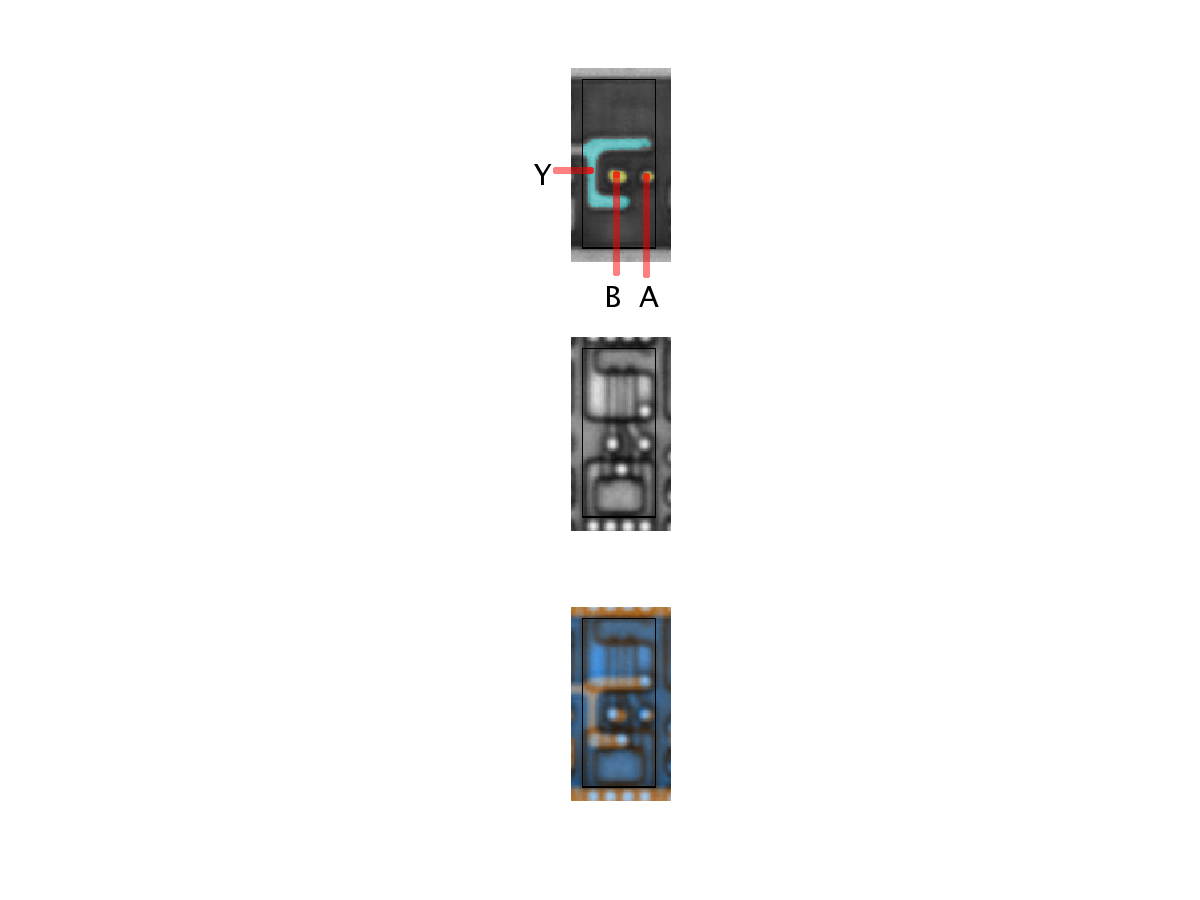2-NOR
Binary function: Y = ~(A+B)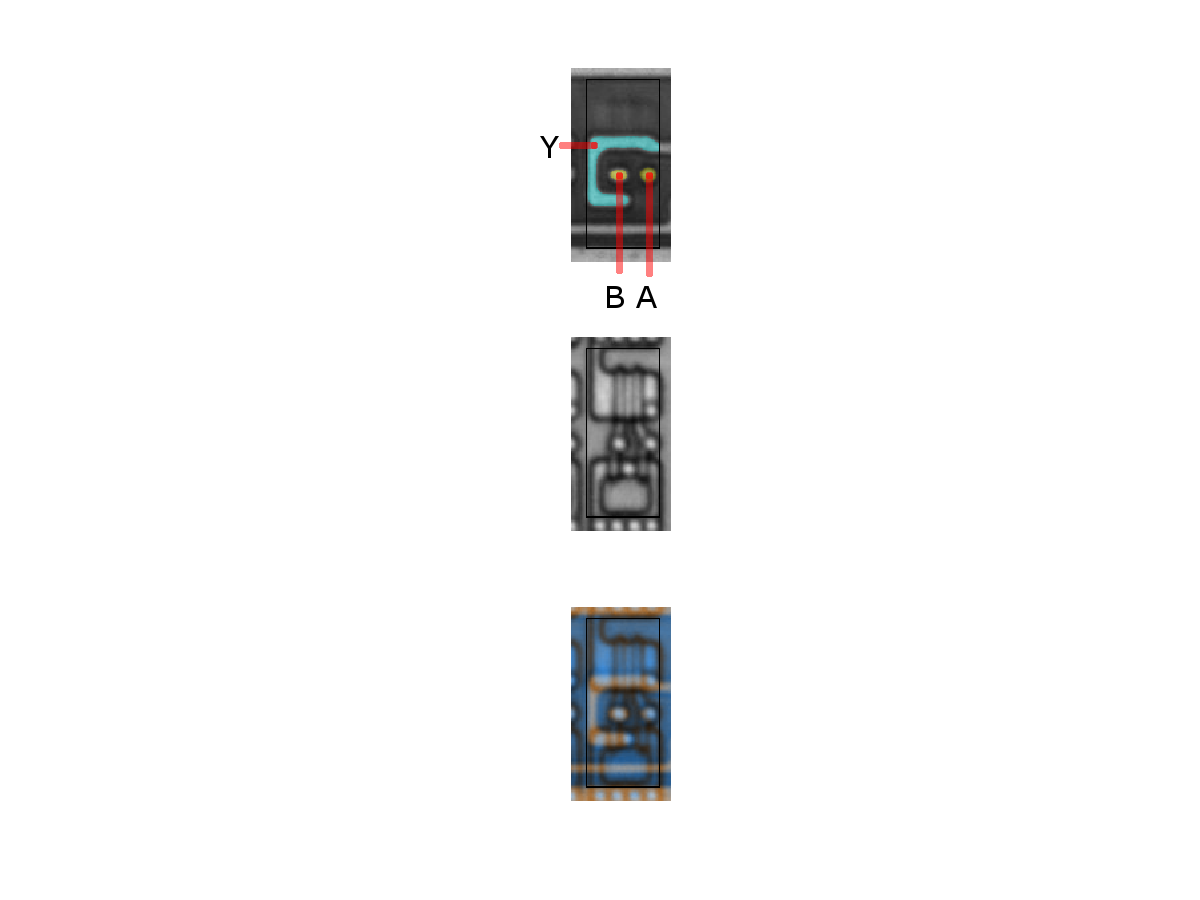2-NOR
Binary function: Y = ~(A+B)2-NOR
Binary function: Y = ~( A+B )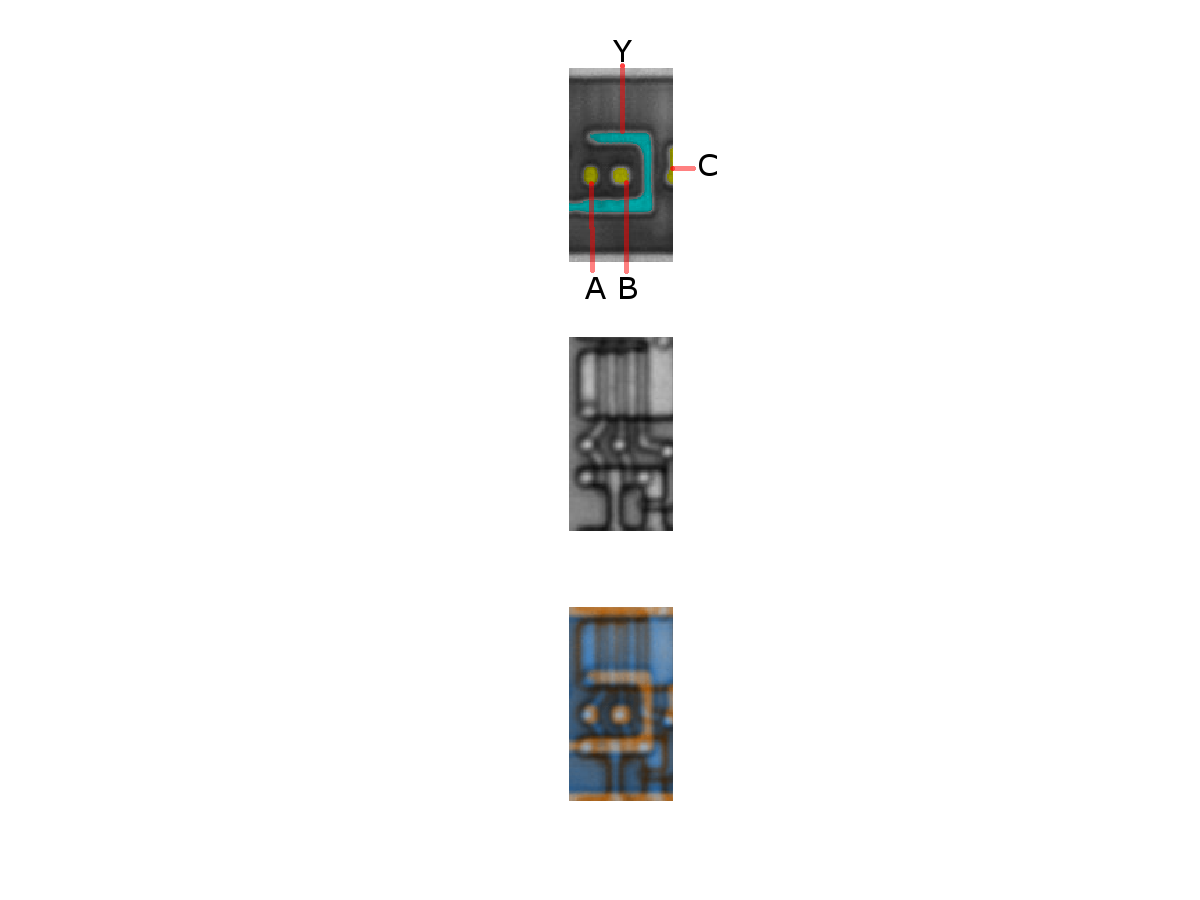3-NOR
Binary function: Y = ~( A+B+C )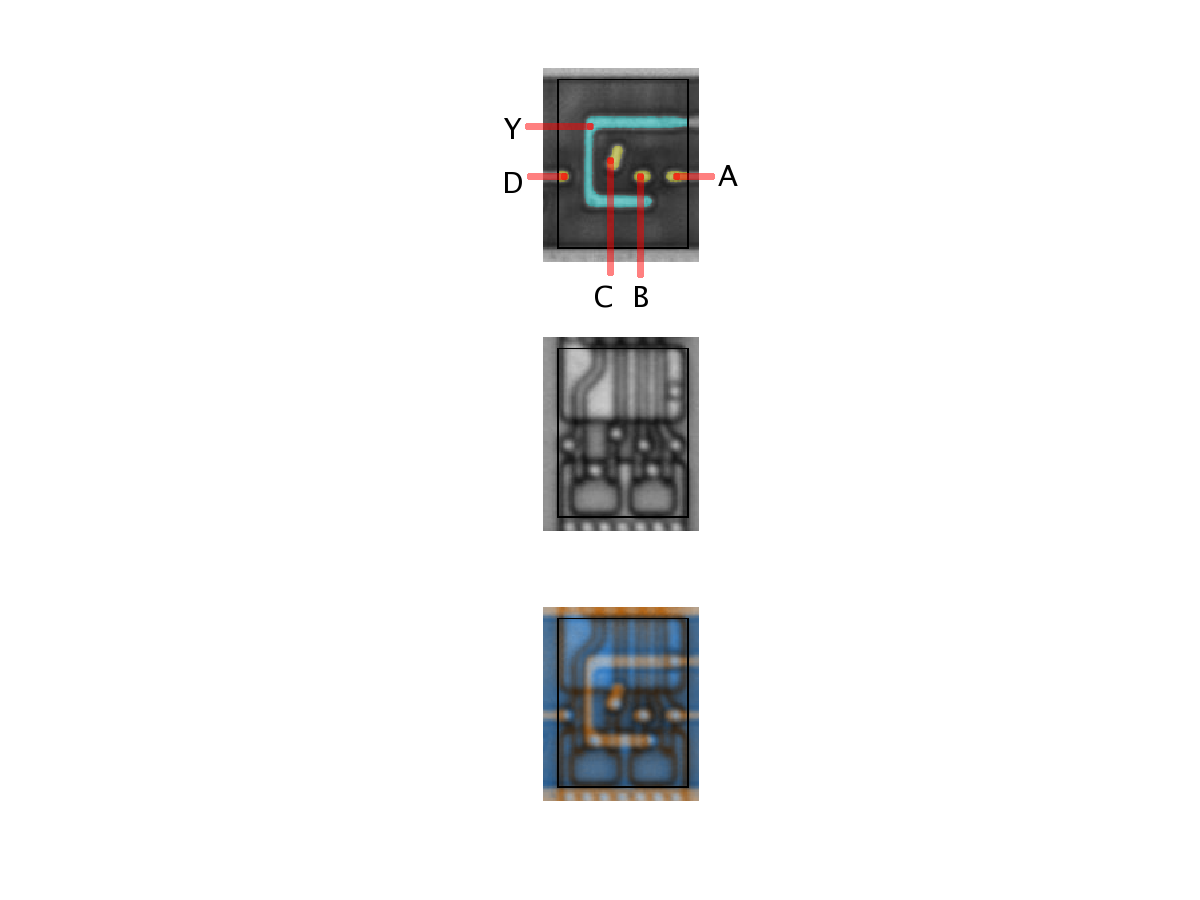4-NOR
Binary function: Y = ~(A+B+C+D)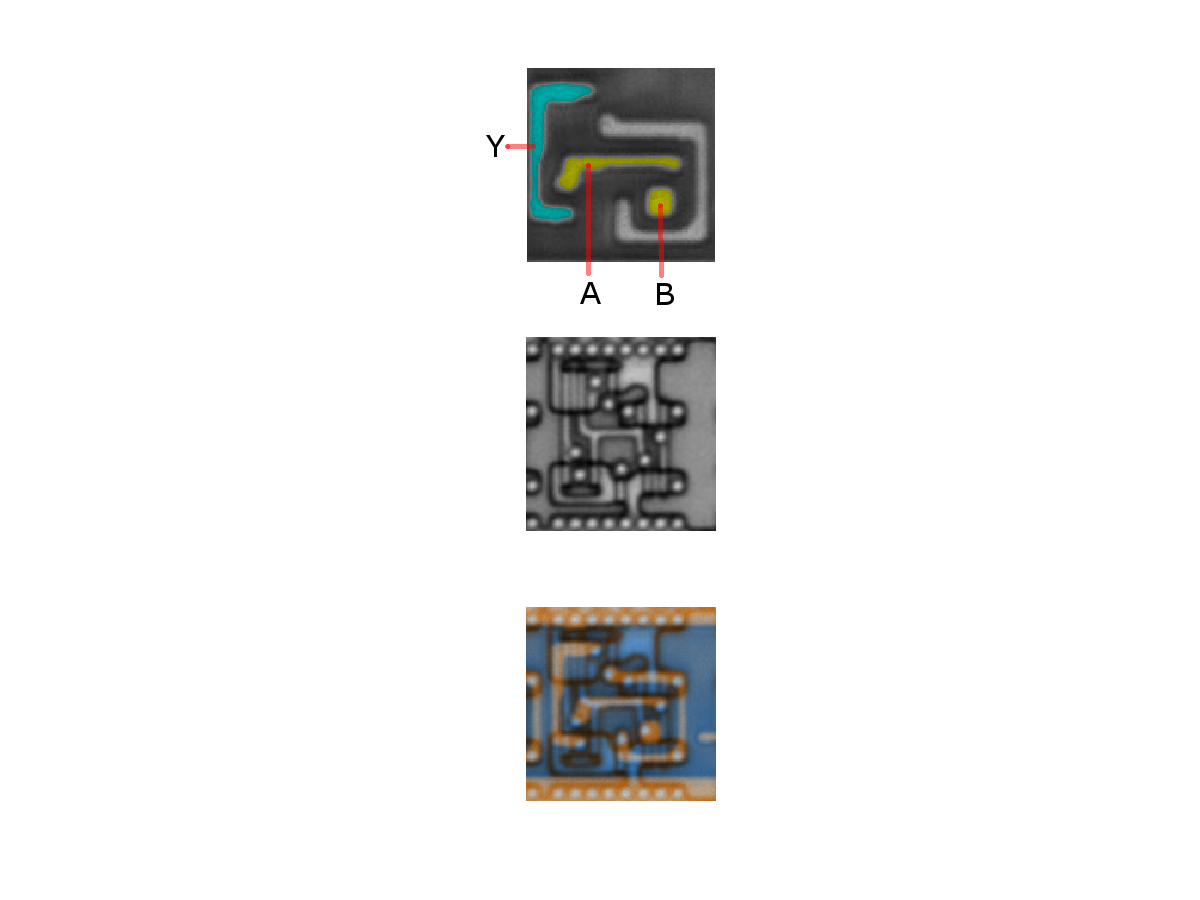2-XNOR
Binary function: Y = ~( A XOR B )2-XOR
Binary function: Y = A XOR B

# AO(I)/OA(I)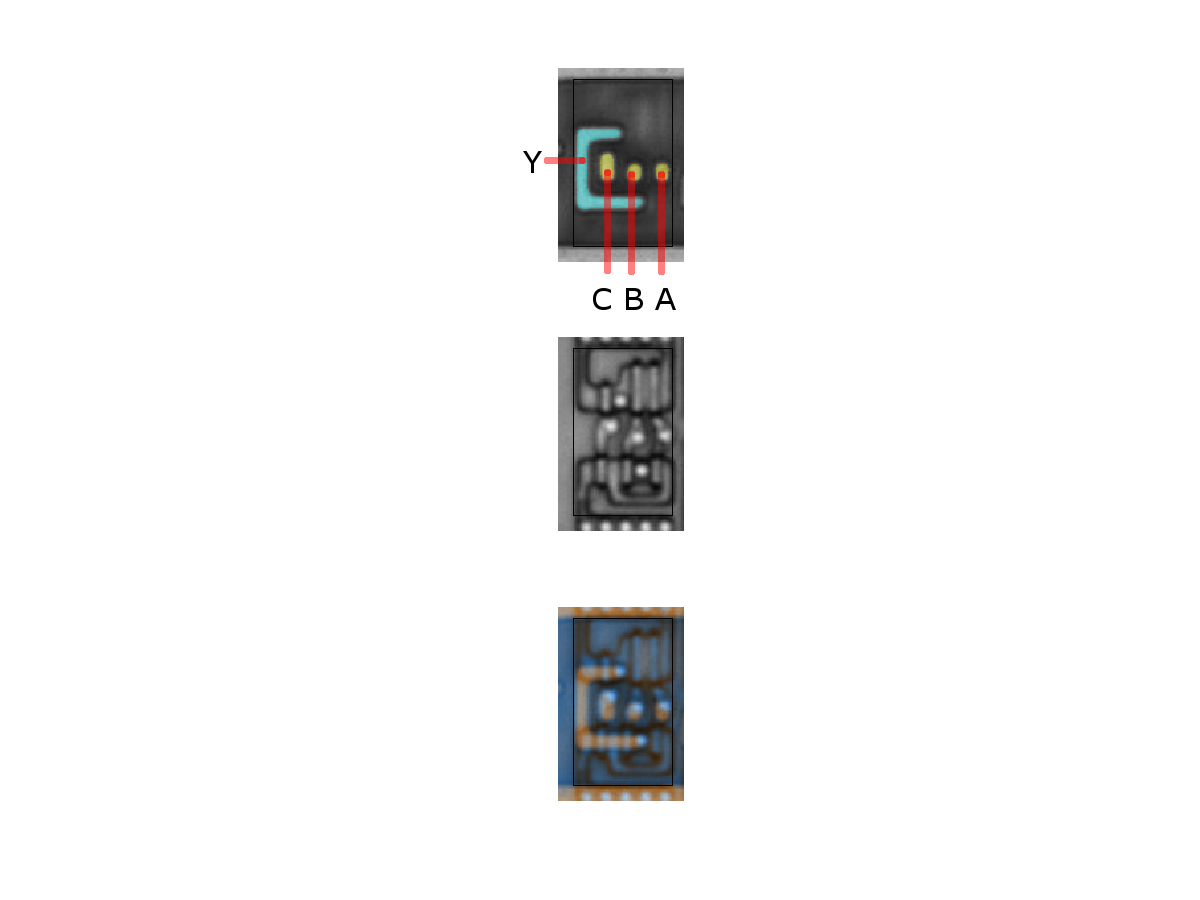21-OAI
Binary function: Y = ~( (A+B)C )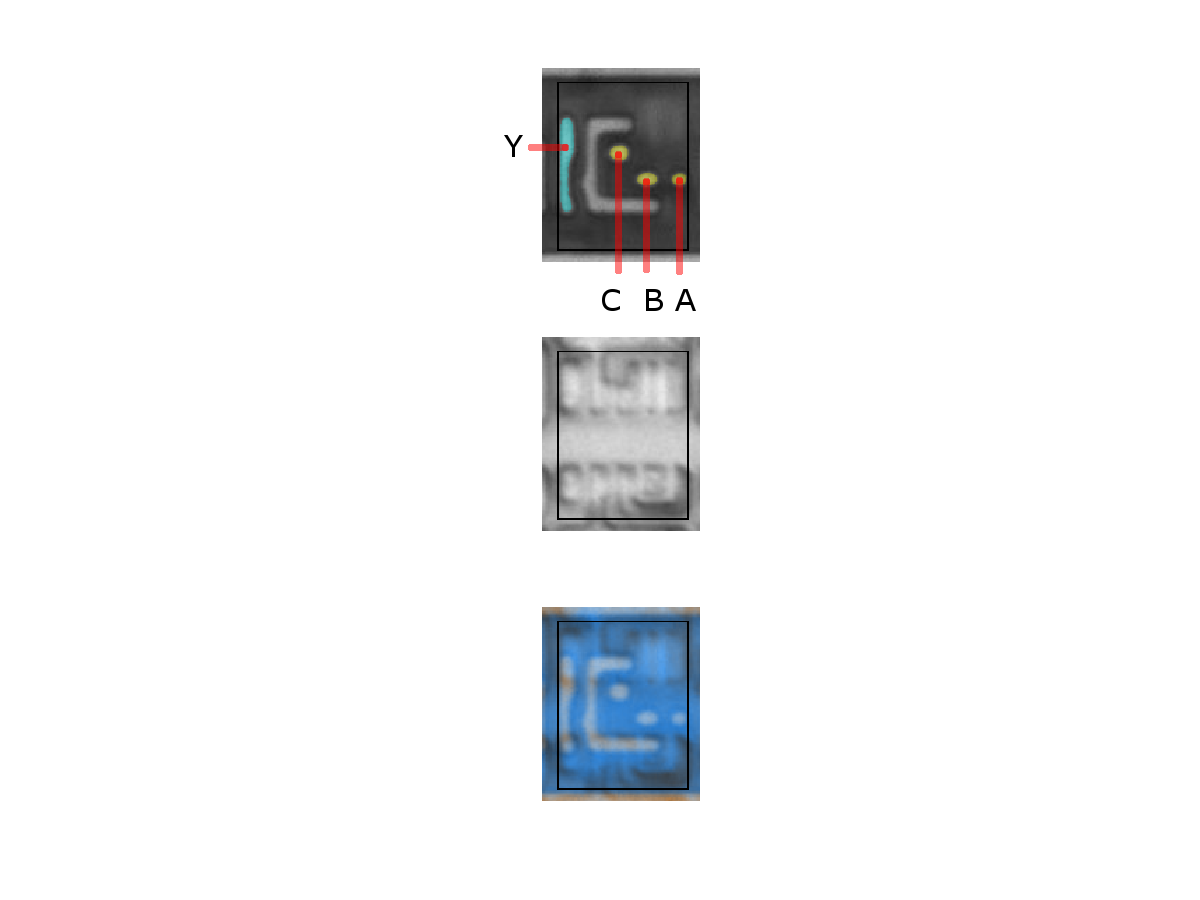21-OA
Binary function: Y = (A+B)C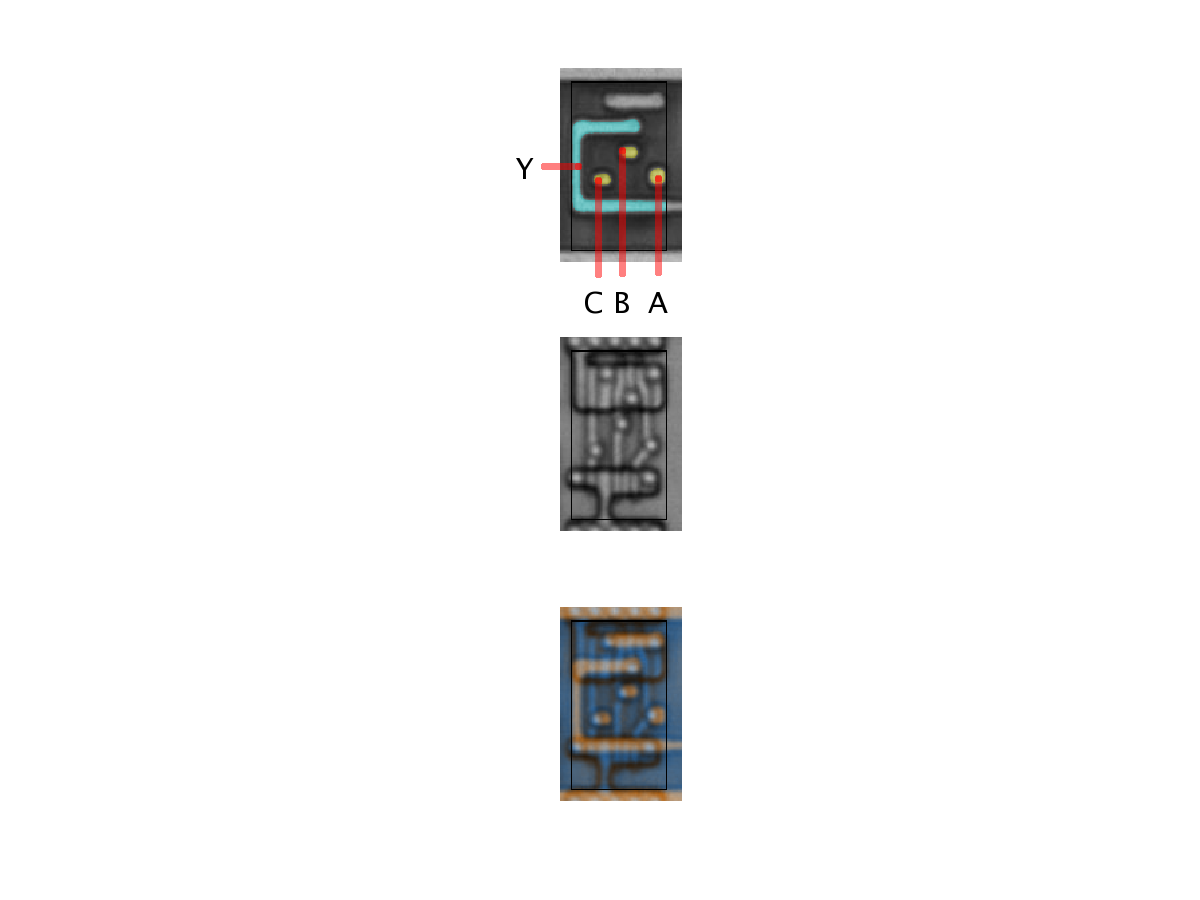21-AOI
Binary function: Y = ~( AB + C)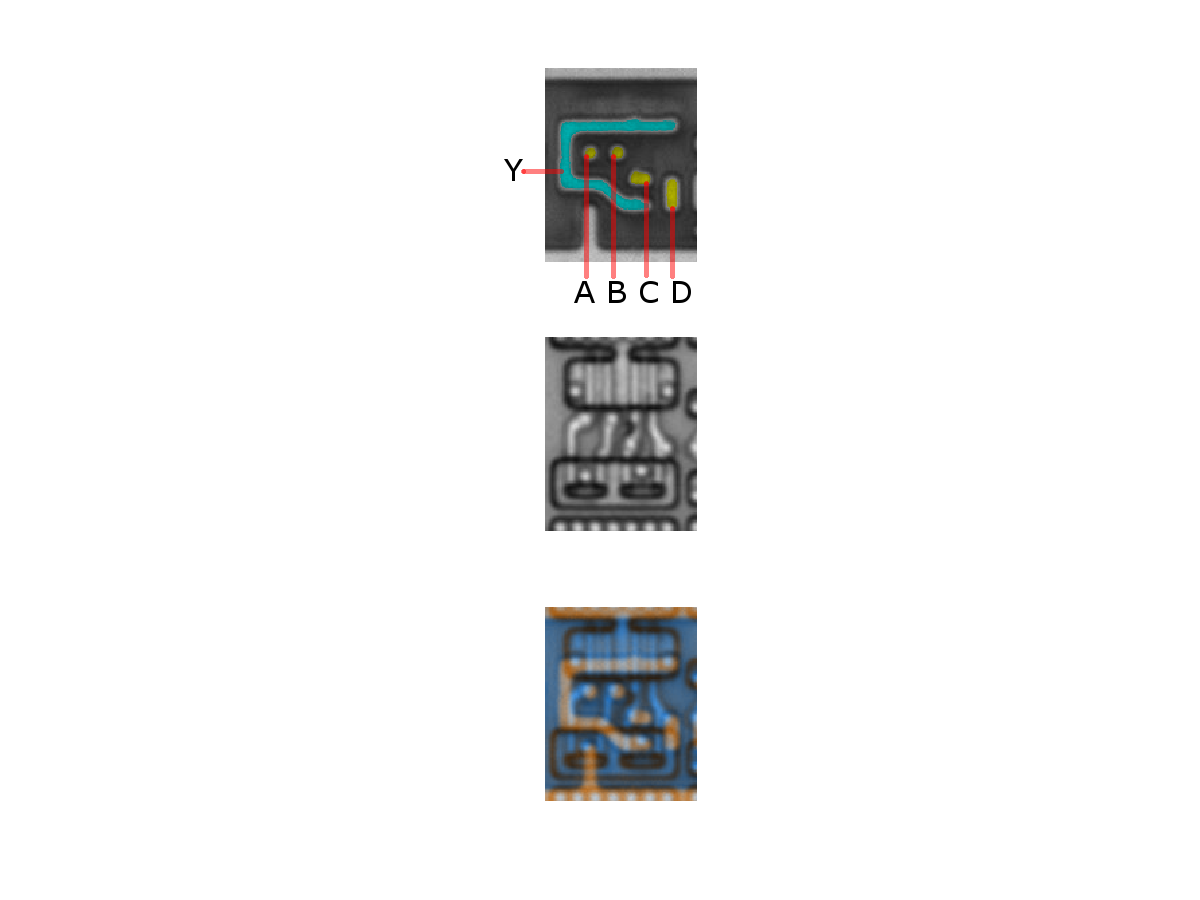22-OAI
Binary function: Y = ~( (A+B)(C+D) )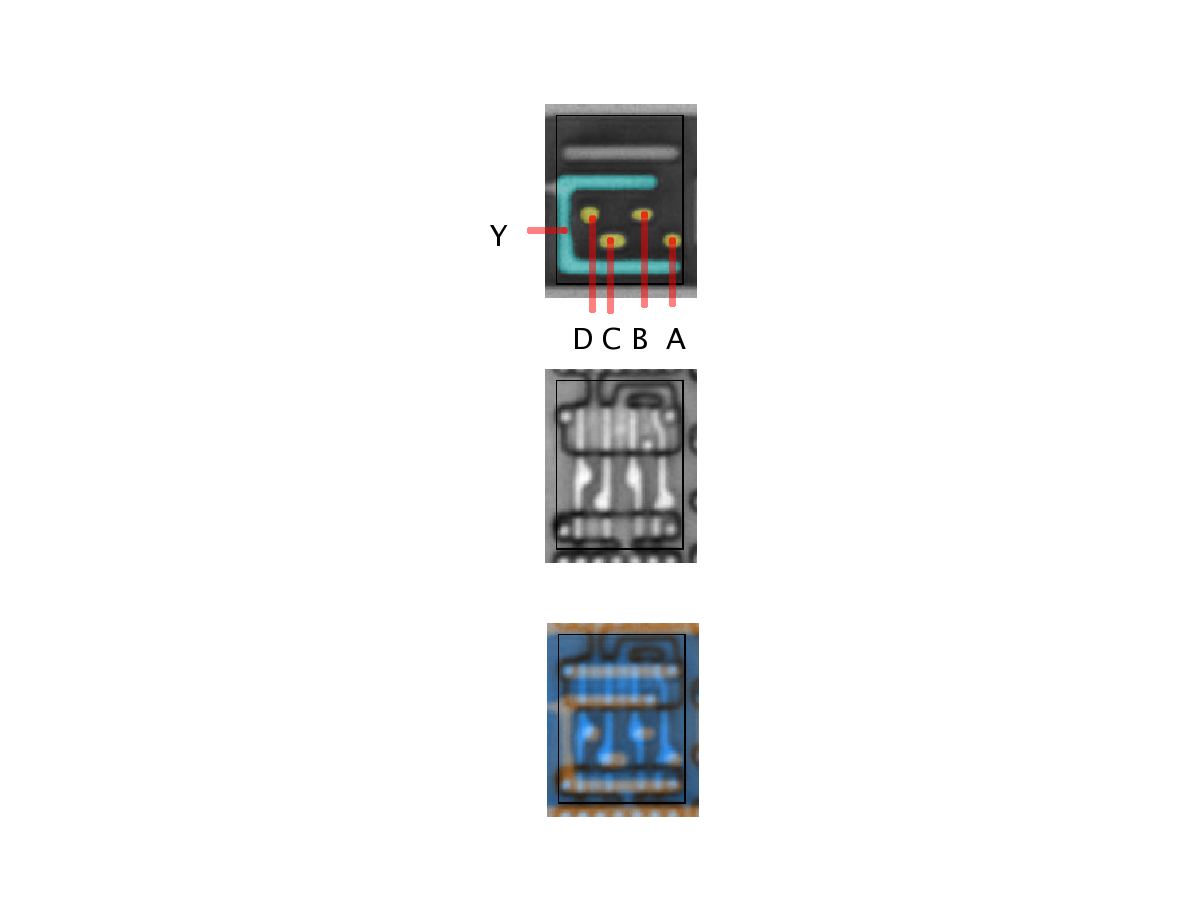22-AOI
Binary function: Y = ~( (AB)+(CD) )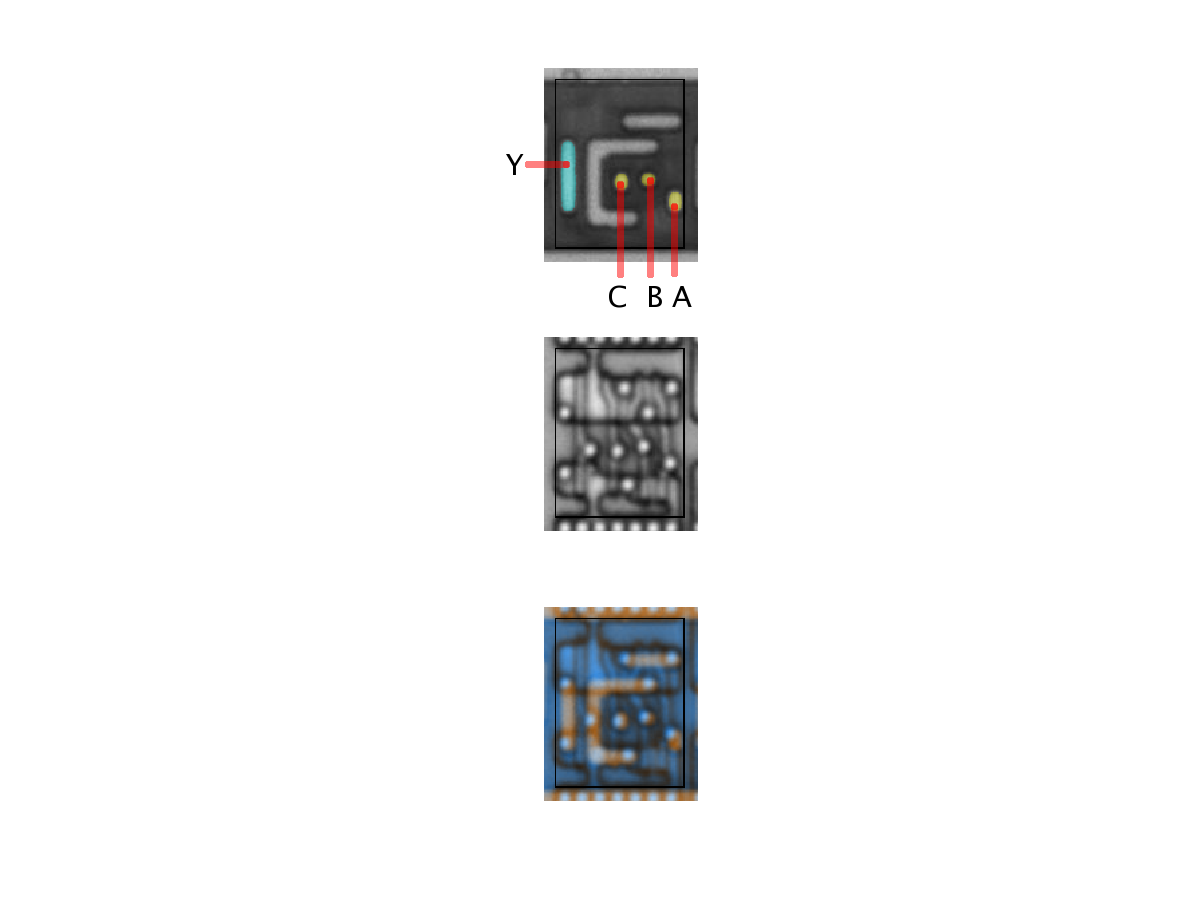21-AO
Binary function: Y = AB + C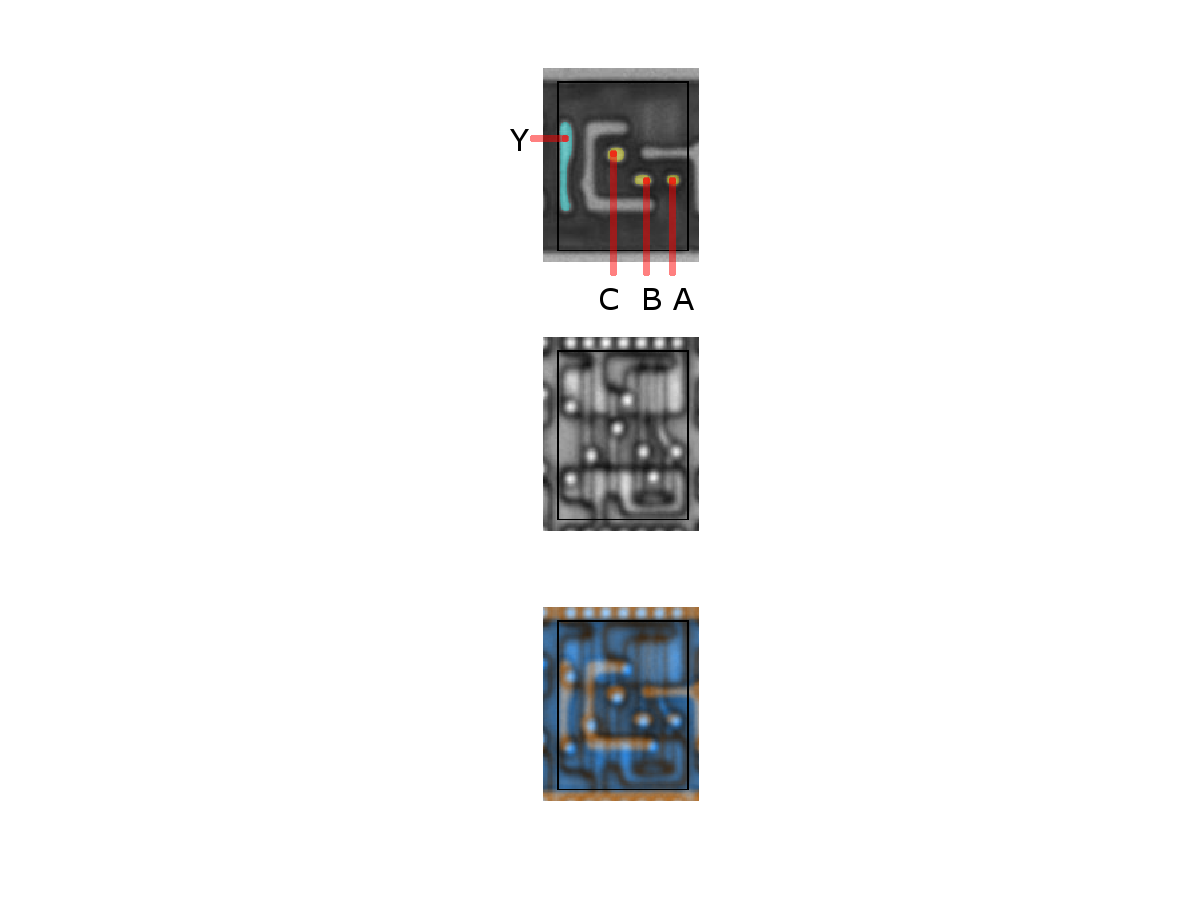21-OA
Binary function: Y = (A+B)C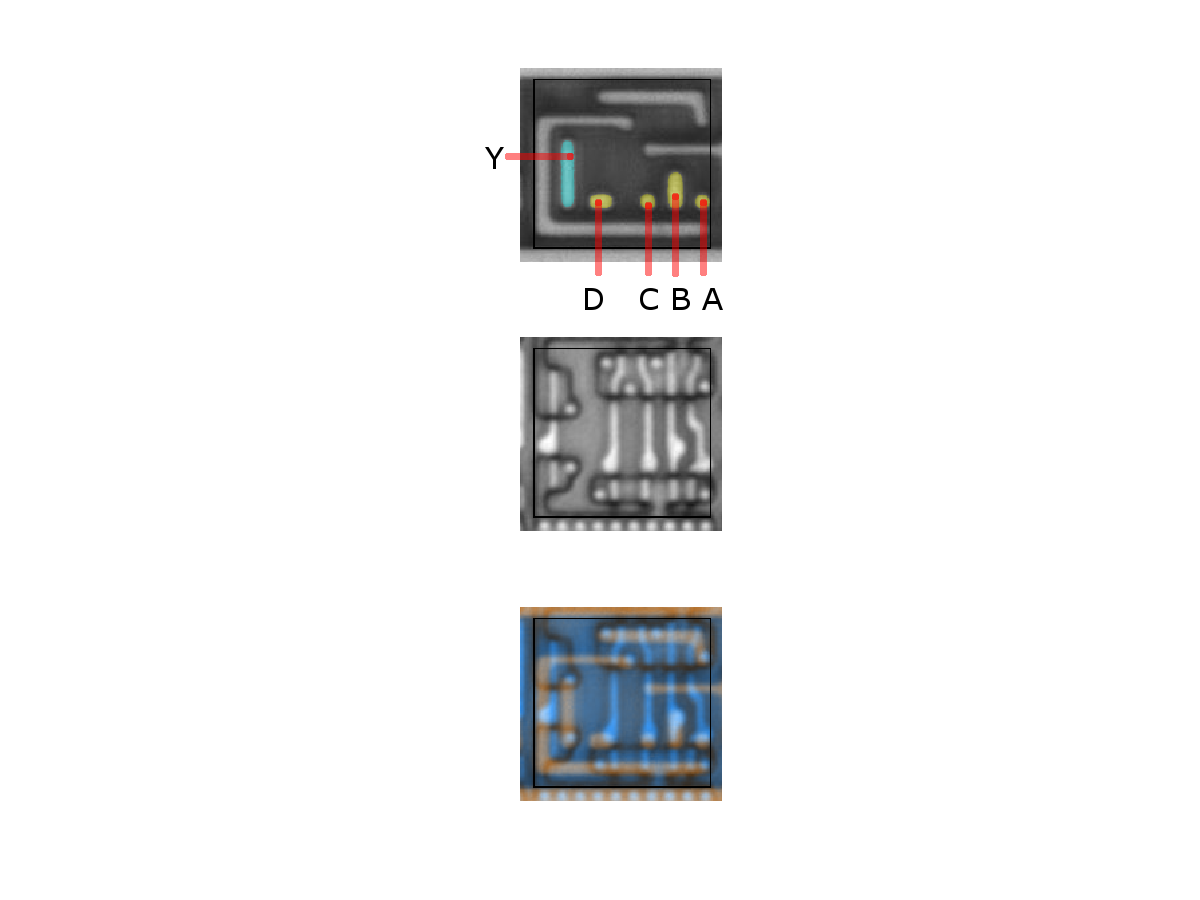22-AO
Binary function: Y = AB + CD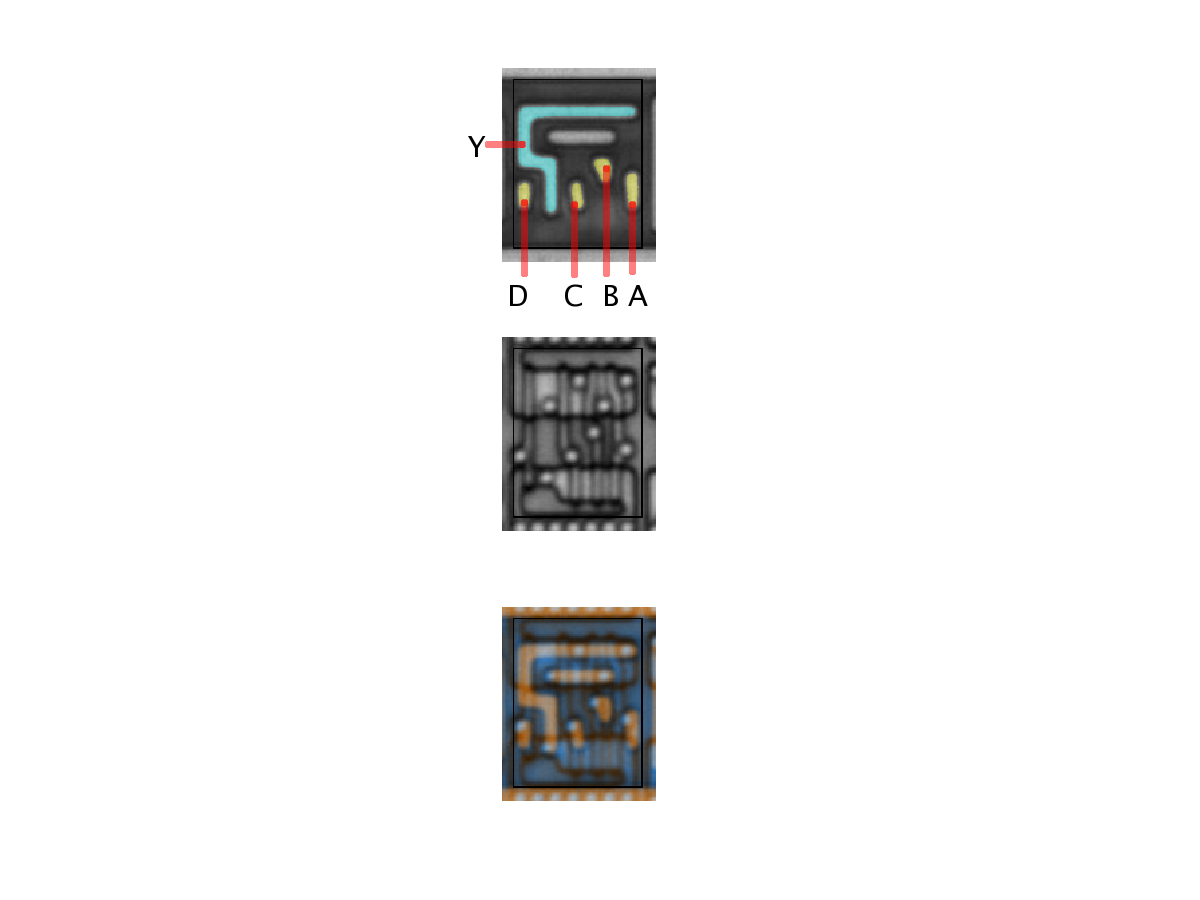31-AOI
Binary function: Y = ~( ABC + D)31-AO
Binary function: Y = ABC + D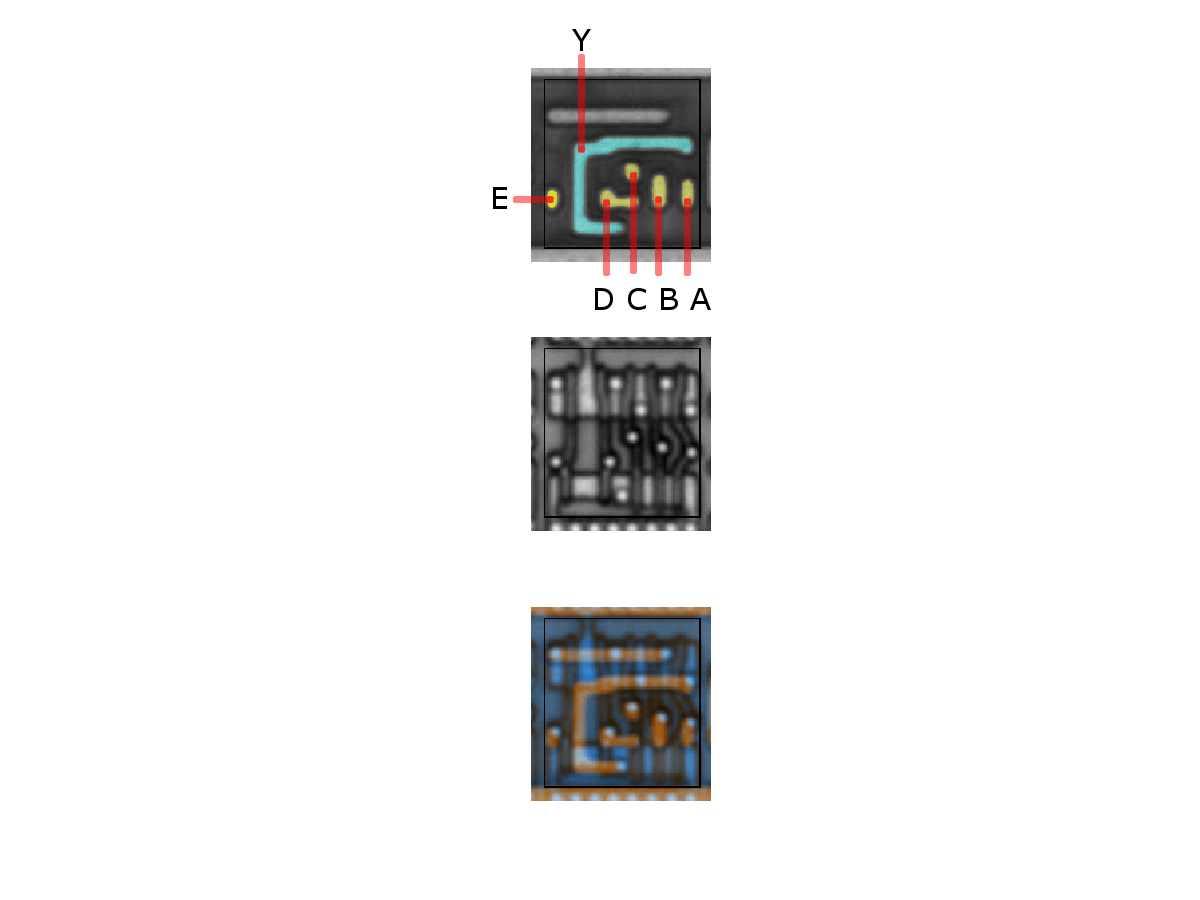32-AOI
Binary function: Y = ~( ED + ABC )32-OAI
Binary function: Y = ~( (A+B+C)(E+D) )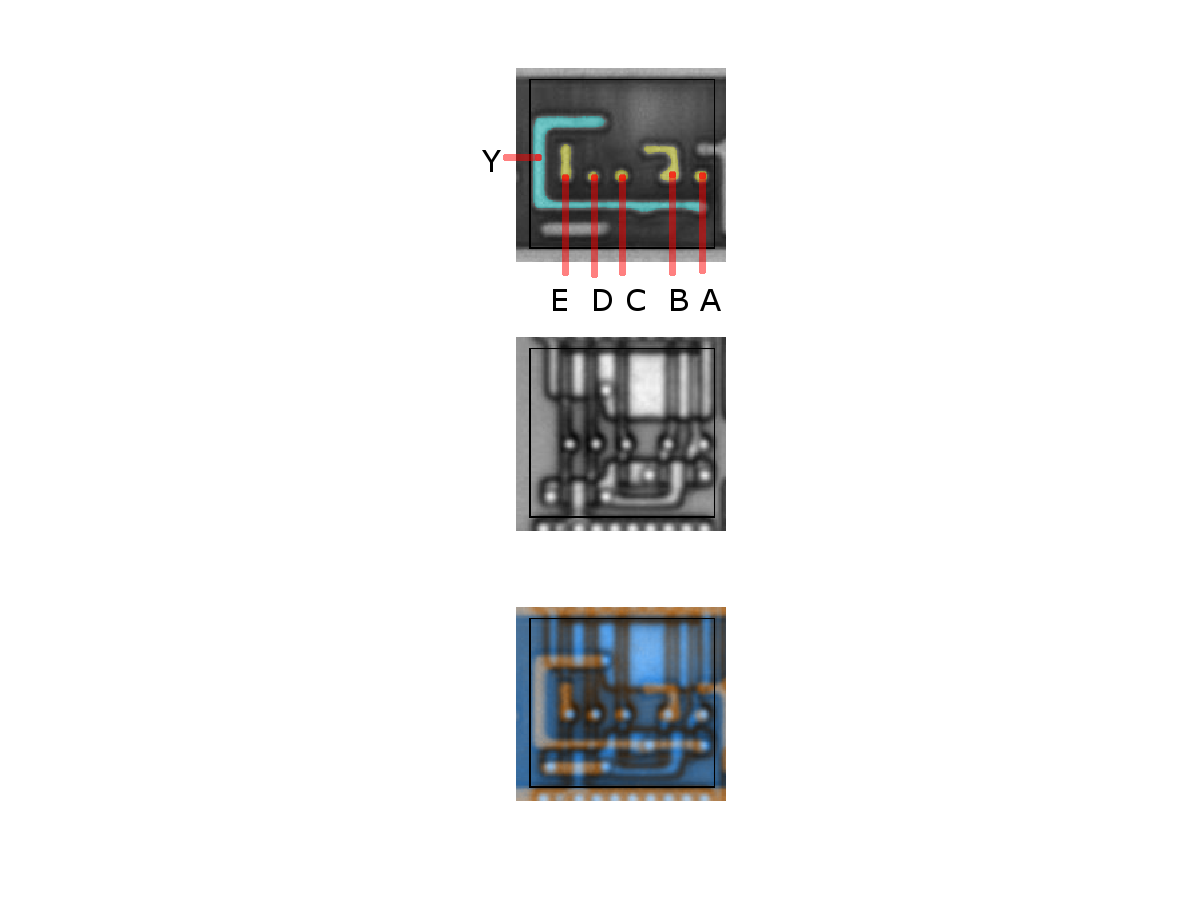32-OAI
Binary function: Y = ~( (A+B+C)(D+E) )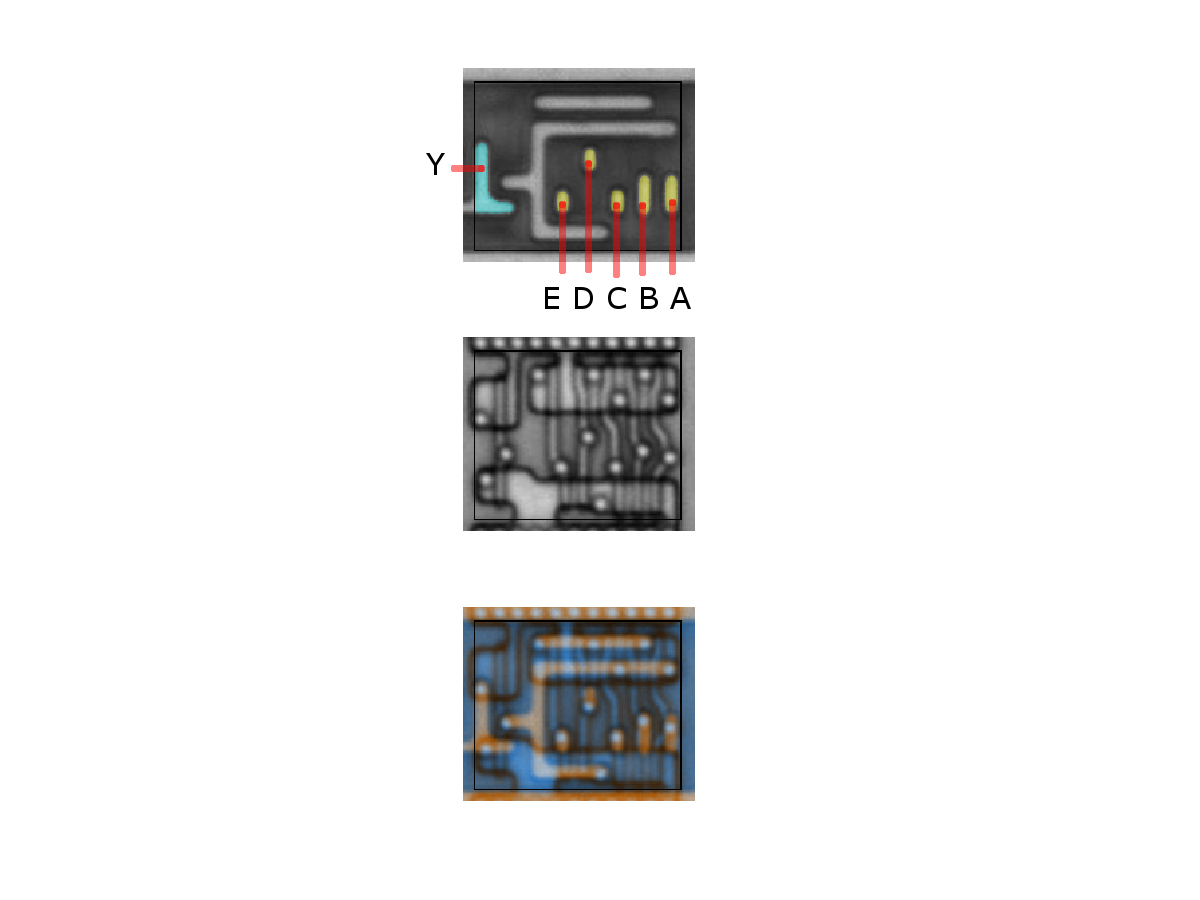32-AO
Binary function: Y = ABC + DE13-OAI
Binary function: Y = ~( C(A+B+D) )32-AO
Binary function: Y = ABC + DE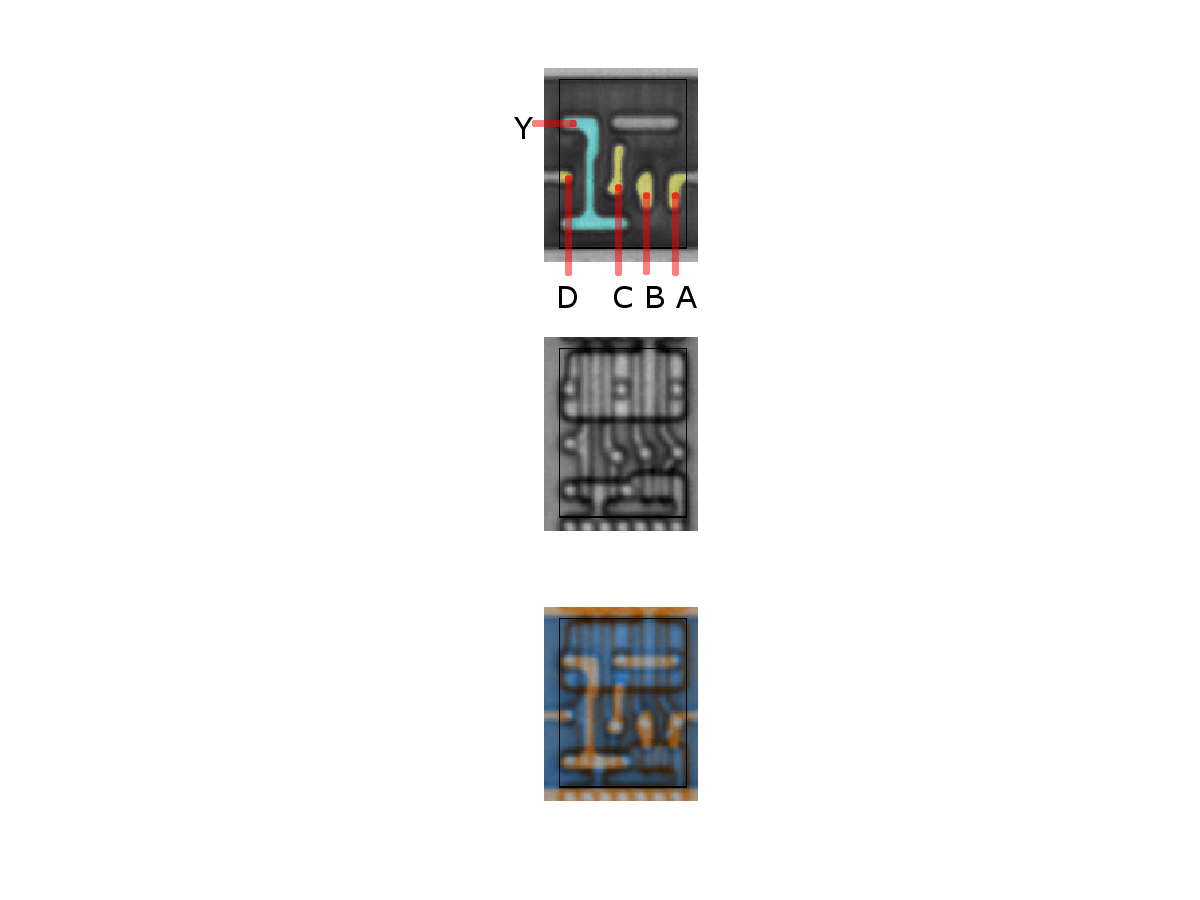211-AOI
Binary function: Y = ~( AB + C + D )211-OAI
Binary function: Y = ~( (A+B)CD )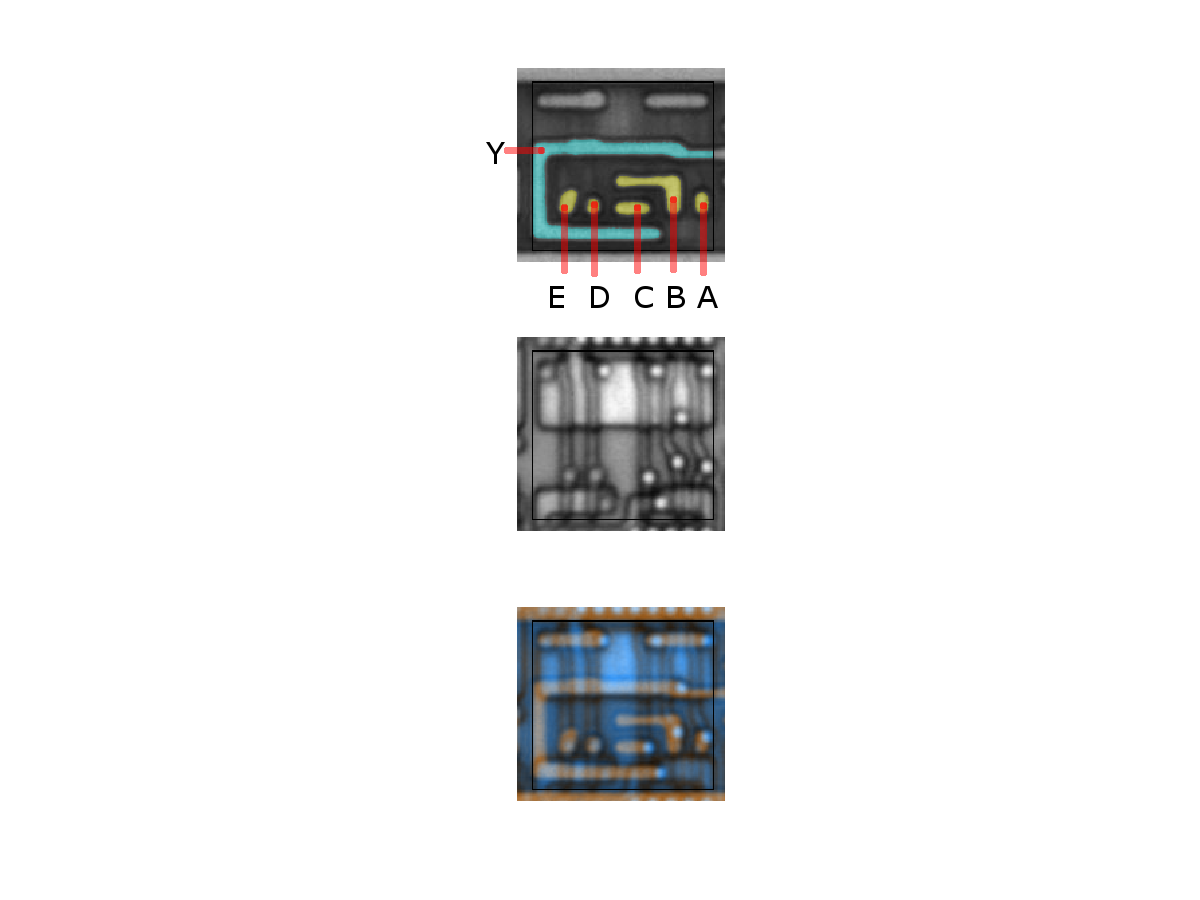212-AOI
Binary function: Y = ~(AB + C + DE)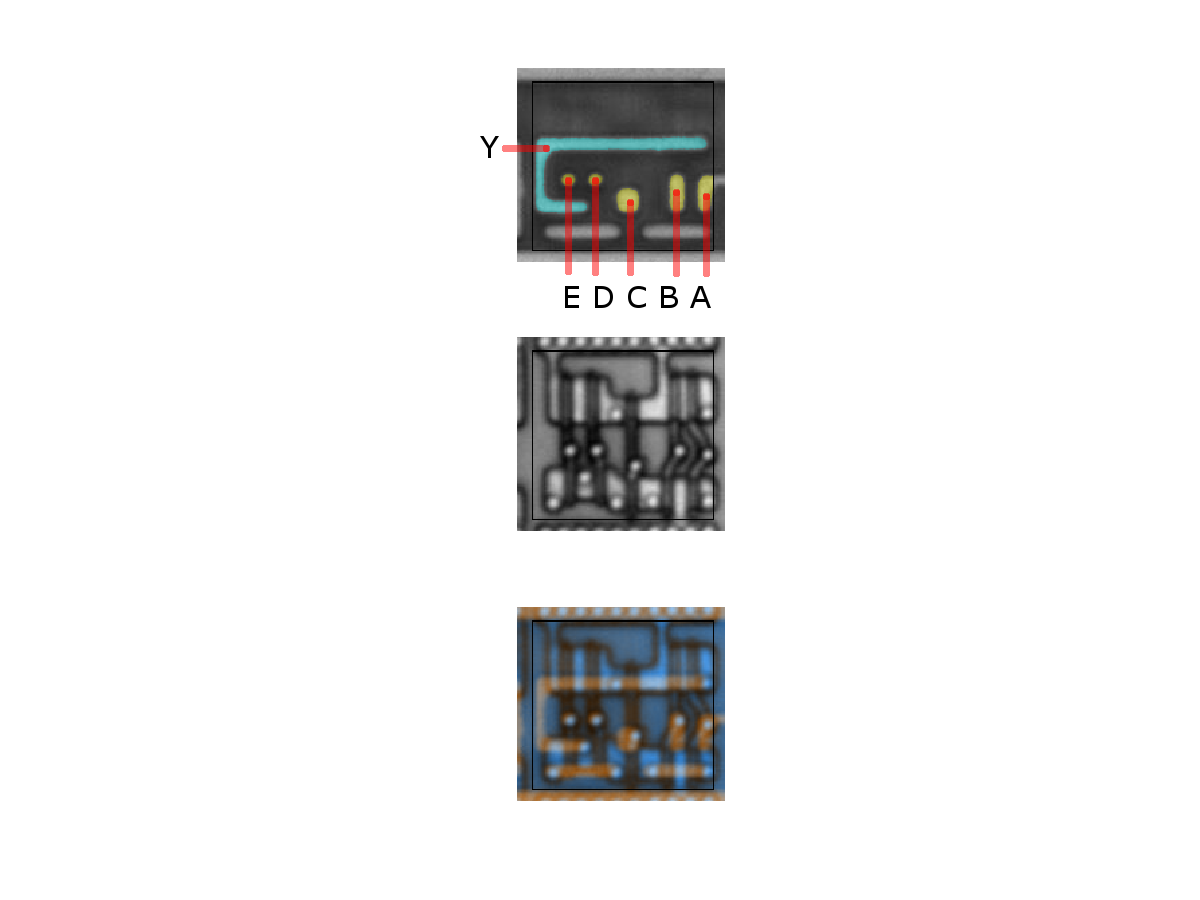212-OAI
Binary function: Y = ~( AB + C + D )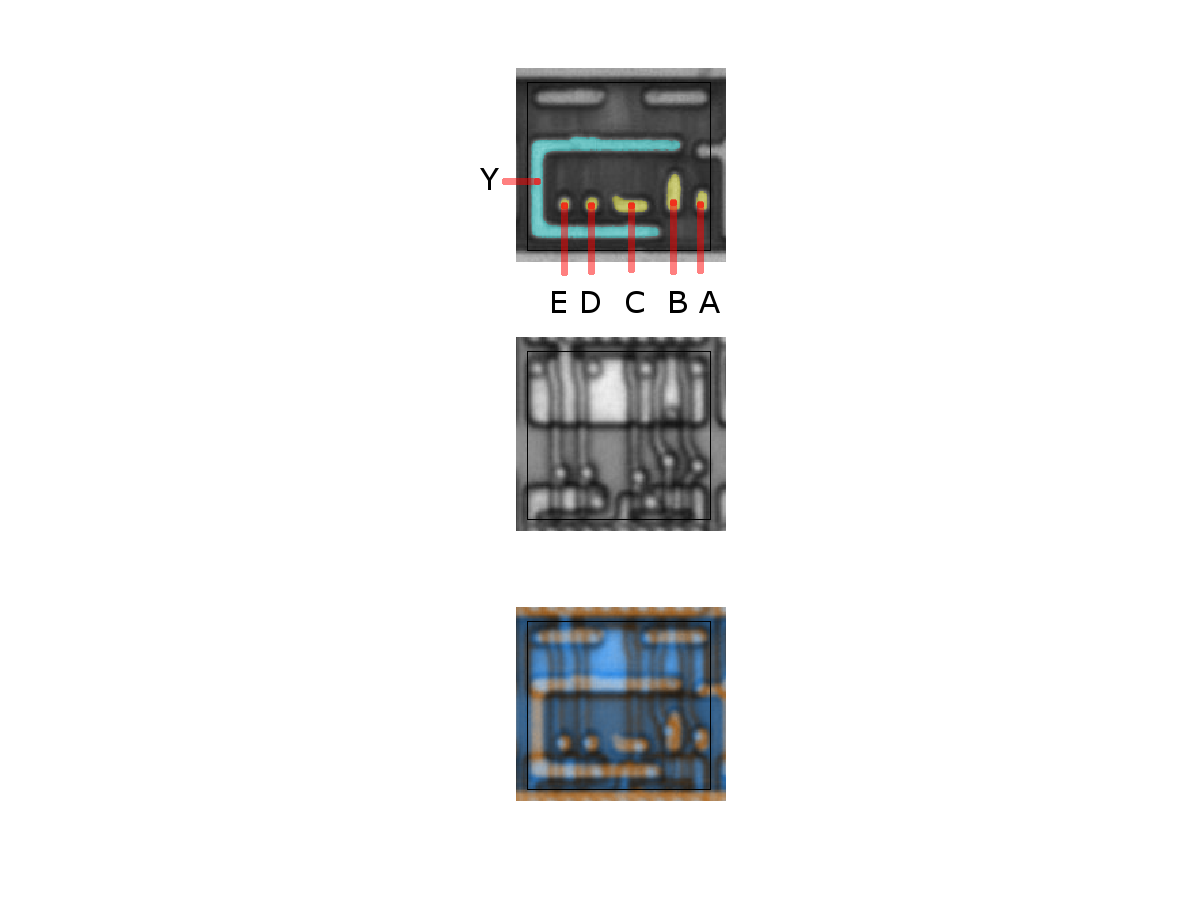212-AOI
Binary function: Y = ~( AB + C + DE )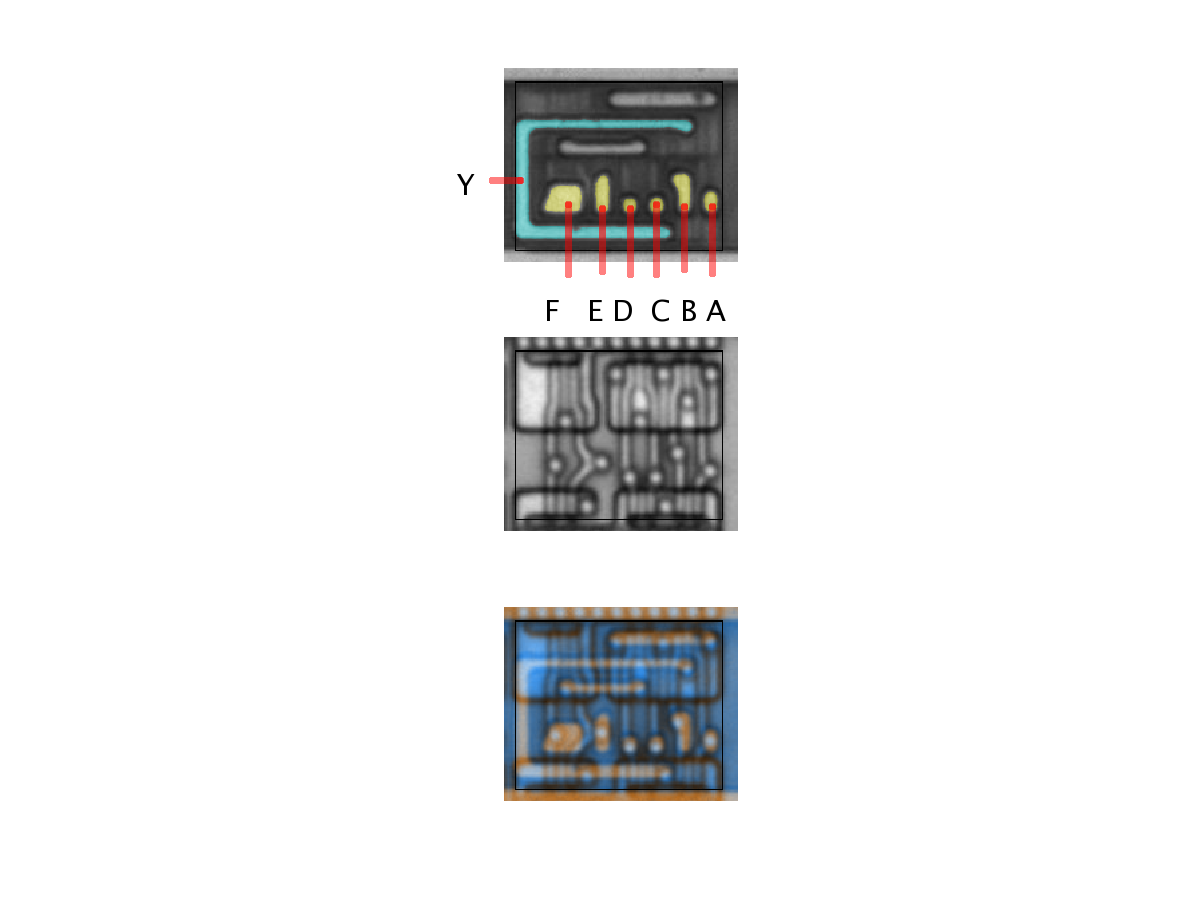222-AOI
Binary function: Y = ~(AB + CD + EF)unknown
Binary function: Y = ???

# LatchesLatch with immediate reset
Binary function: D: in, Q: outLatch with immediate reset
Binary function: D: in, Q: outLatch (samples at clk=high)
Binary function: D:in, Q:out

# Flip-FlopsD-Q flip-flop w/o reset
Binary function: d=data input, q=data output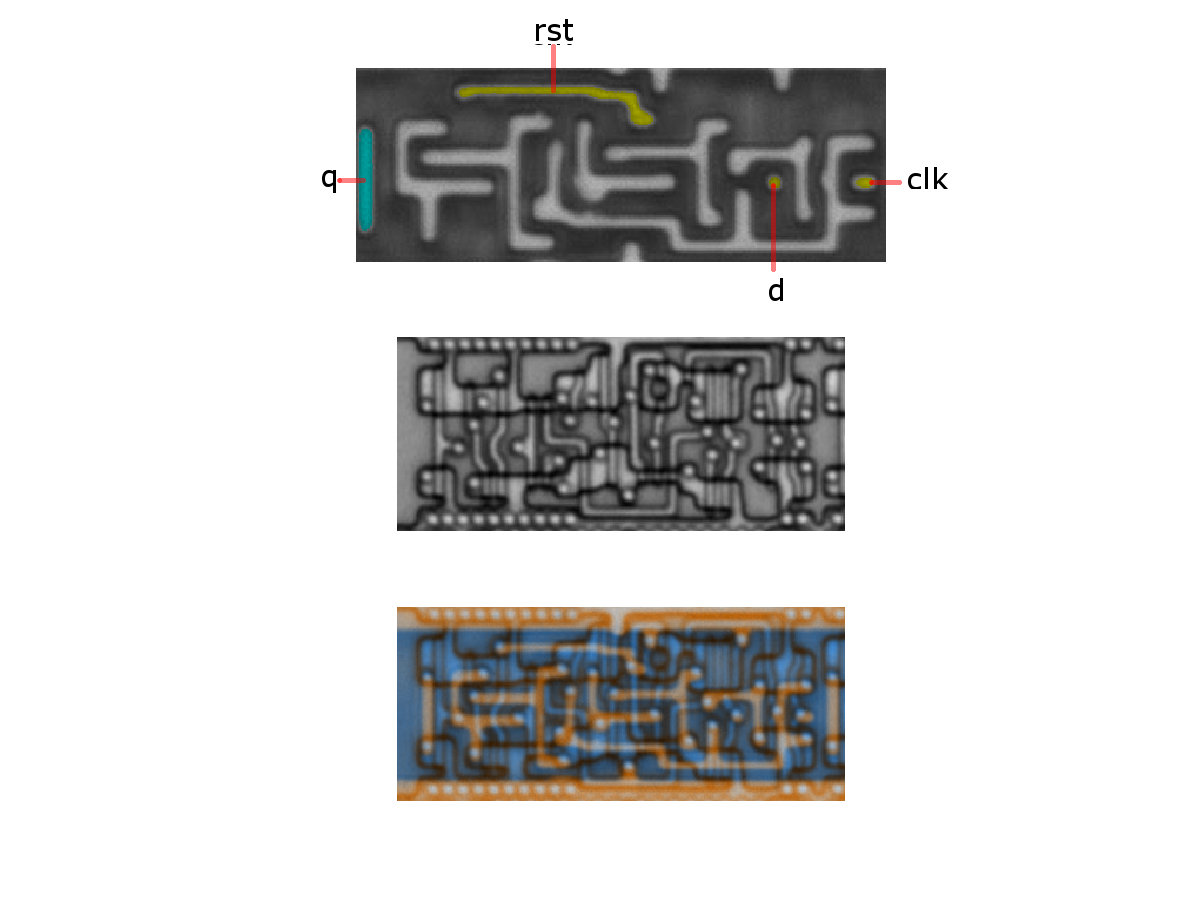D-Q flip-flop w/ delayed reset
Binary function: d=data in, q=data out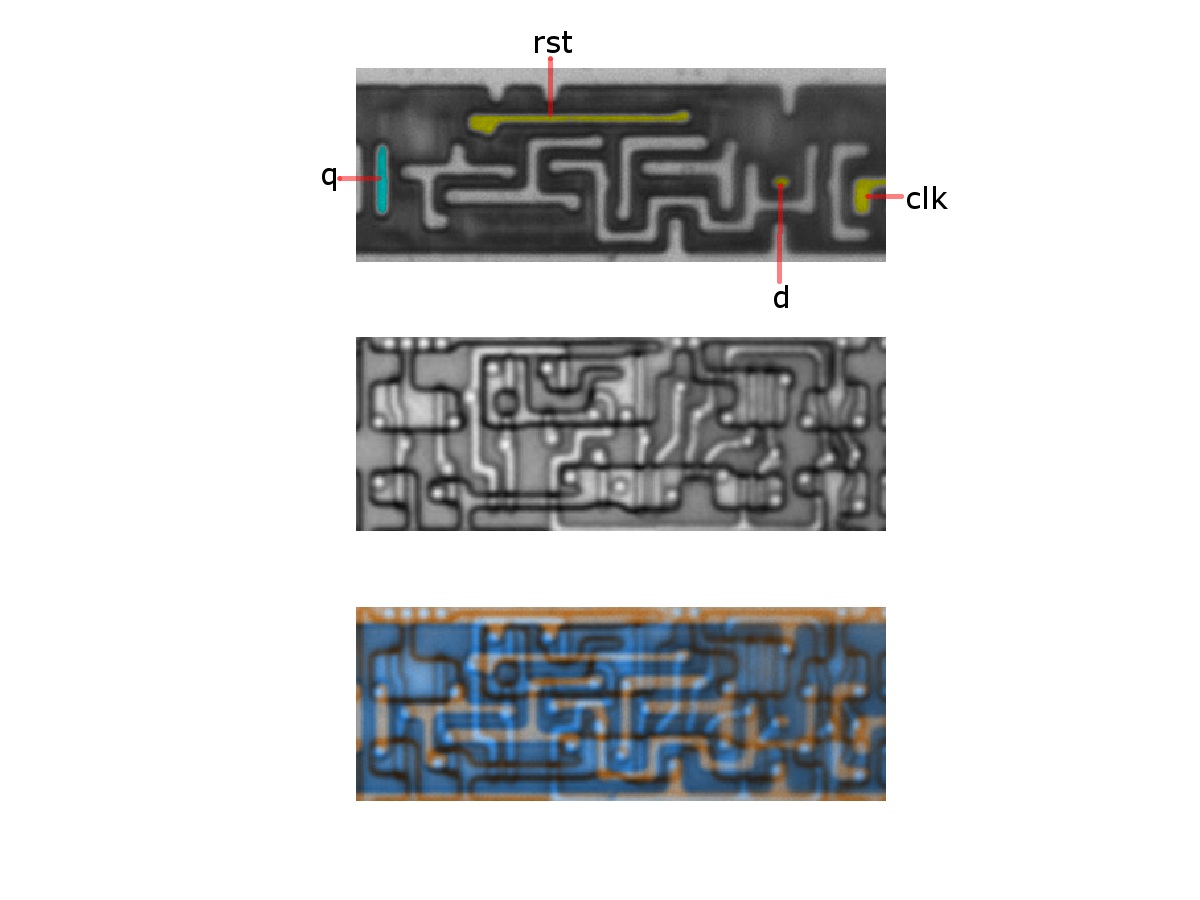D-Q flip-flop w/ immediate reset
Binary function: d=data input, q=data output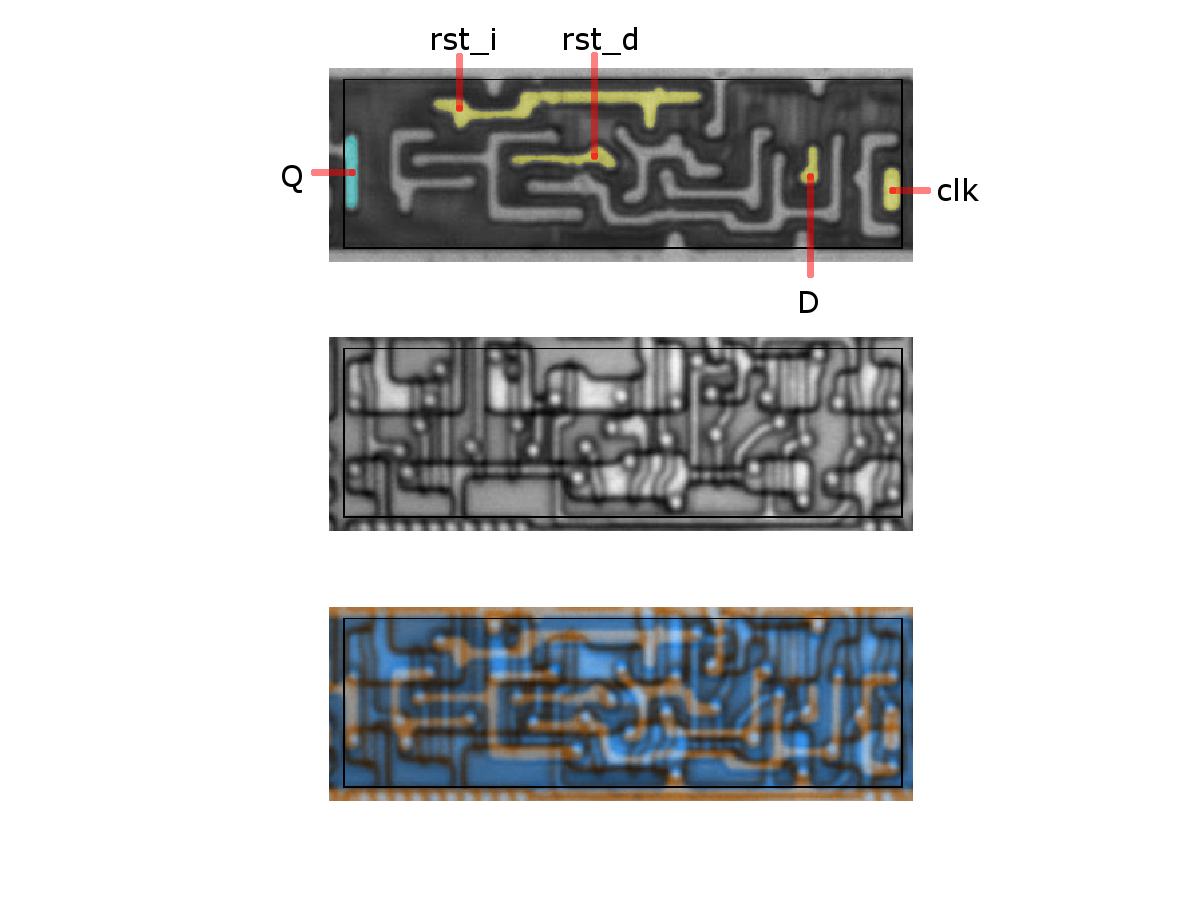Flip-Flop with immediate and delayed reset
Binary function: D: in, Q: outsome complex flip-flop
Binary function: ???

# Isolationisolation
Binary function: noneisolation
Binary function: noneisolation
Binary function: none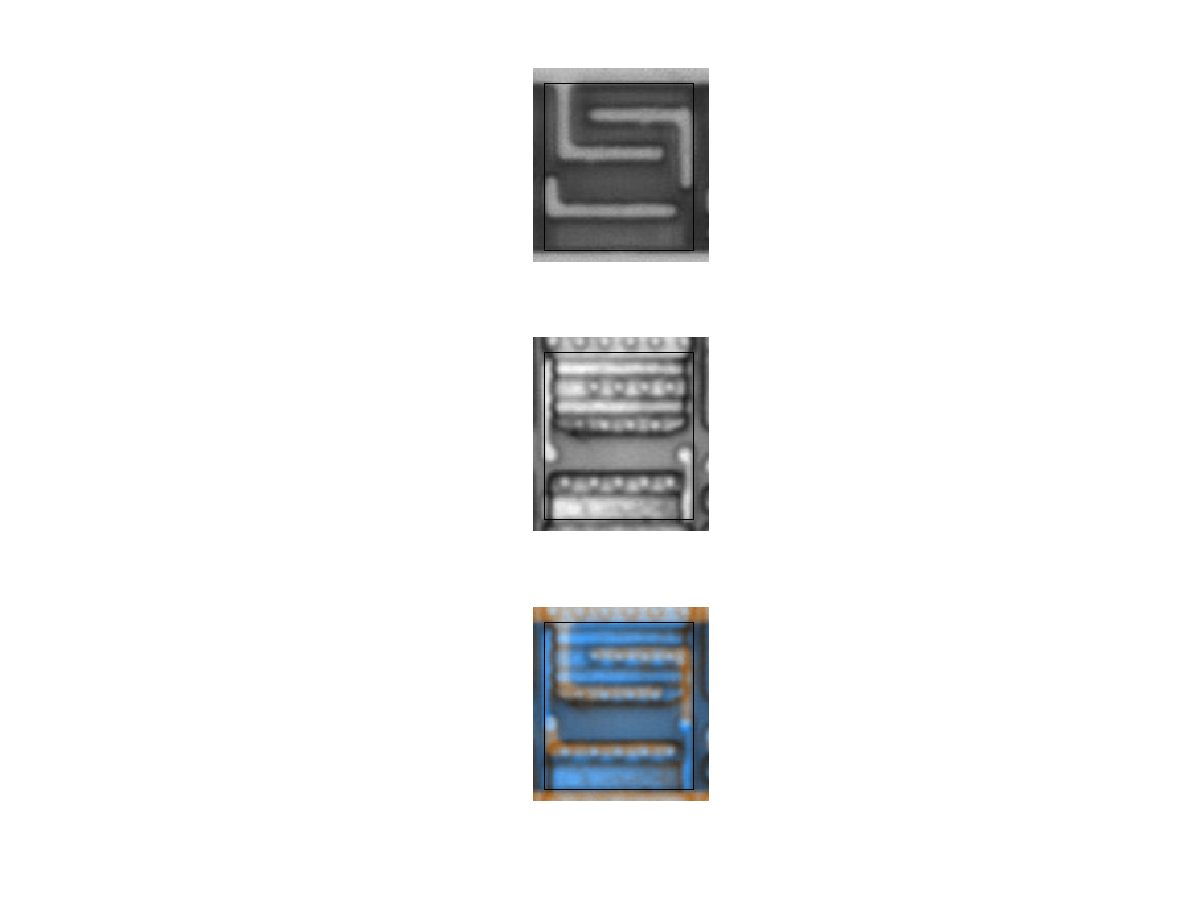isolation
Binary function: noneisolation
Binary function: none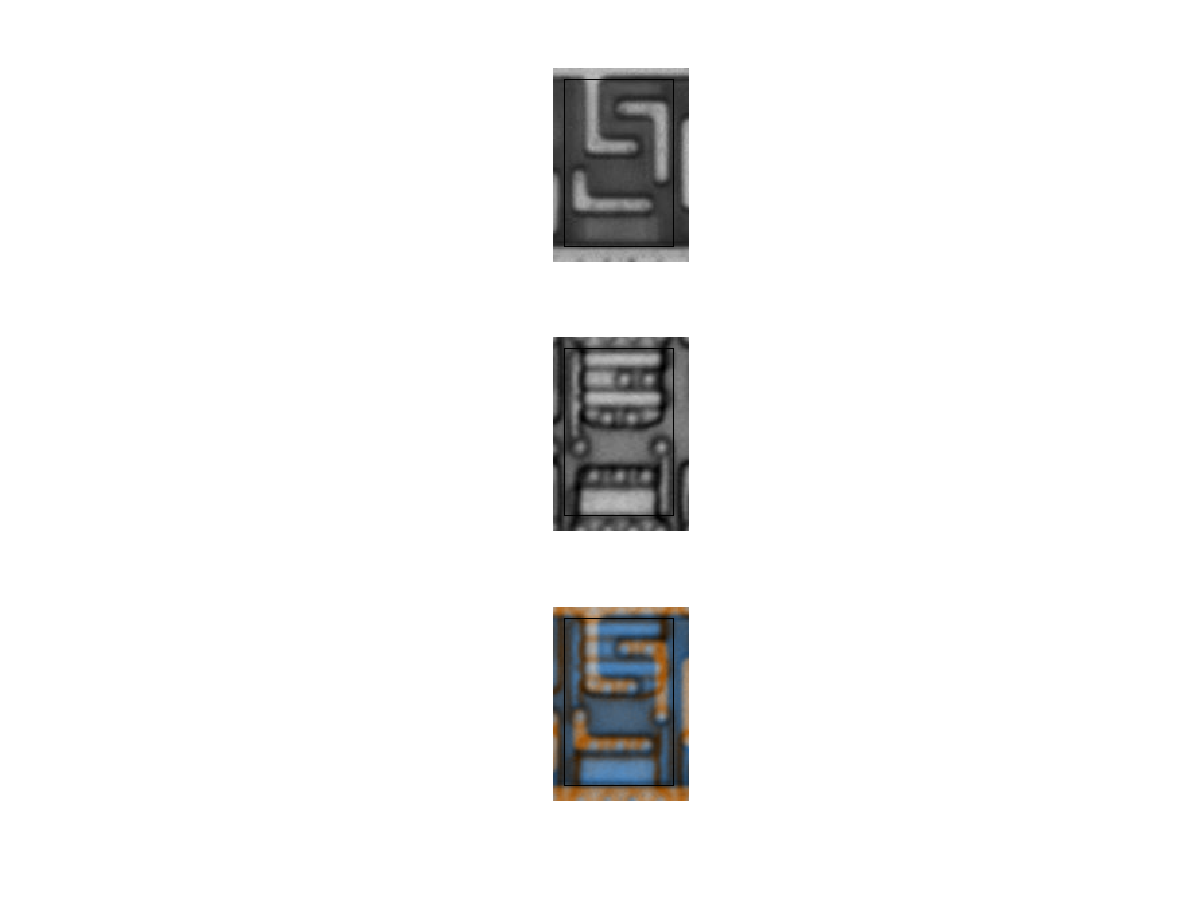isolation
Binary function: none

# Misc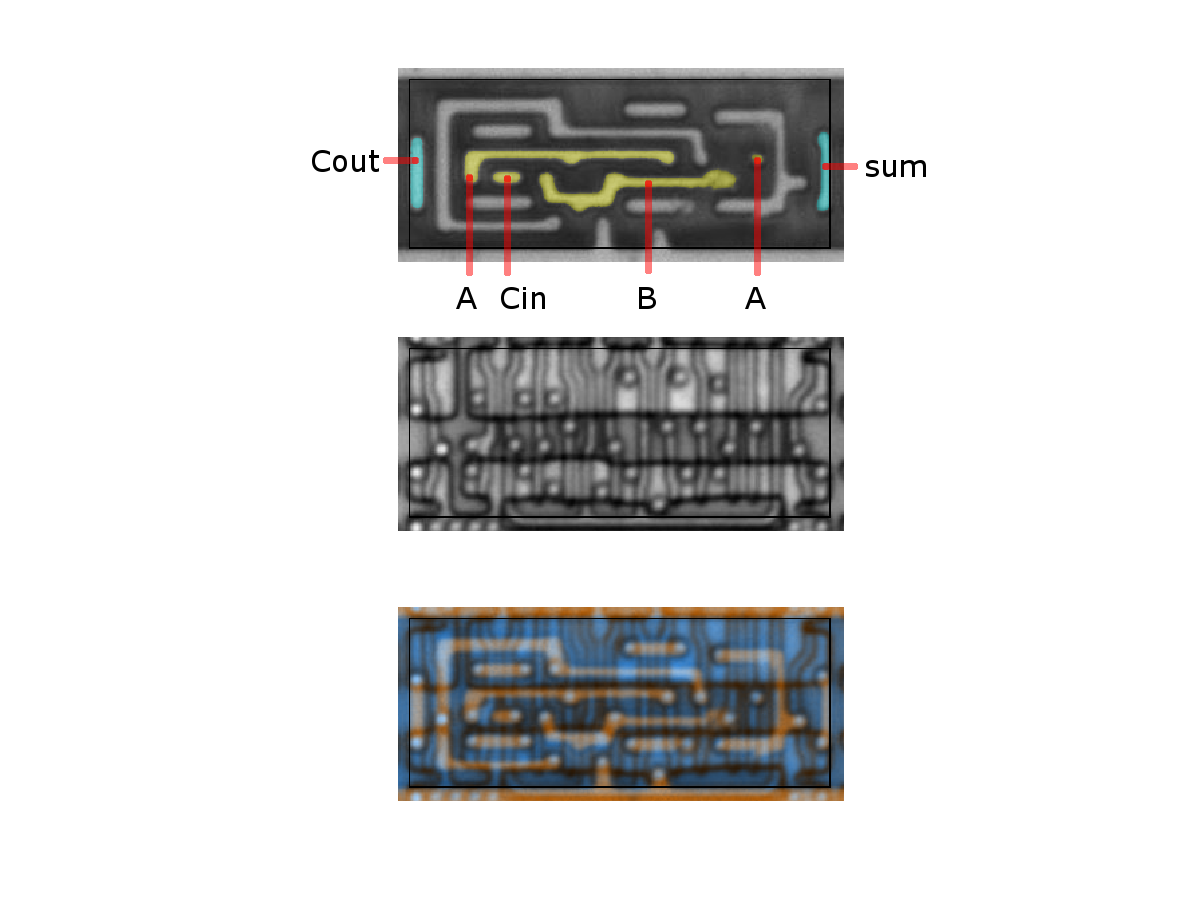Binary function: